• ## LR分解

千次阅读 2018-04-13 10:09:19
在数值进行计算时，LR分解不单是最常用的方法之一，还是众多算法的基础。给定方阵矩阵A，通过LR分解将A分解成下三角矩阵L和上三角矩阵U的乘机，成为LR分解，也称LU分解。而我们希望得到对角线是1，如下图所示。而在...
在数值进行计算时，LR分解不单是最常用的方法之一，还是众多算法的基础。
给定方阵矩阵A，通过LR分解将A分解成下三角矩阵L和上三角矩阵U的乘机，成为LR分解，也称LU分解。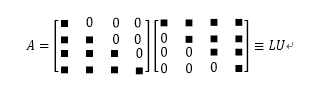而我们希望得到对角线是1，如下图所示。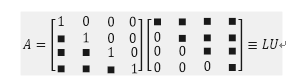而在非方阵Ａ中，我们希望得到如下图。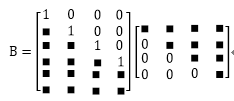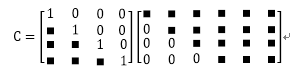一旦完成了LR分解，利用Ｌ和Ｕ的性质，去求行列式的值或者方程组的值。利用LR的上三角矩阵或者下三角矩阵可以减少计算的次数。
该方法的可能性我就不证明了，可以利用矩阵中的黑色点也就是实数的地方进行矩阵相乘，就可以表示原矩阵了。但是，这种方法有一个缺点，如果相乘得到的结果是0的话就说明该矩阵分解出来的L和U是错误的，需要重新计算了。
计算的次数如下所示：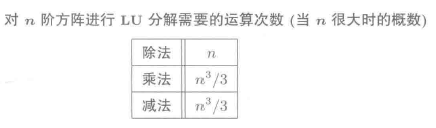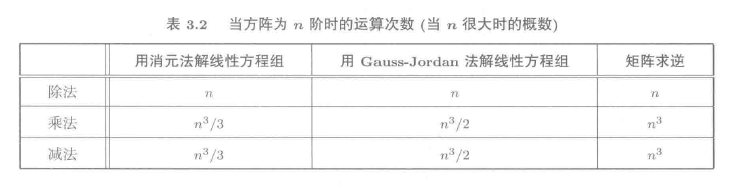求解：
（１）求解线性方程组
将上述的满足转换为线性方程组，通过对角线值为１以及为０的线性方程组进行求解。
（２）利用元素的位置
在这篇证明中，就是通过分析各个元素之前的关系，得到的LR分解的矩阵，详细具体分析地址：点击打开链接
公式如下：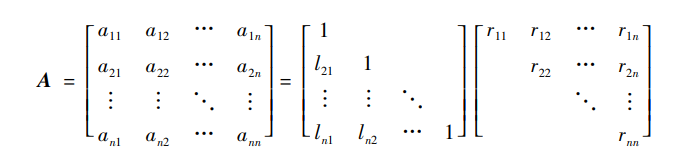求出L矩阵的前 k-1 行和Ｒ矩阵的前 k-1 列元素后，在求k行和k列，具体值如下公式。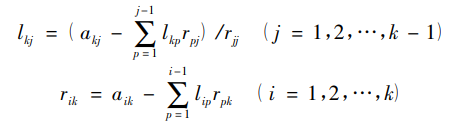应用：
（1）行列式的求解
（2）线性方程组
展开全文• 矩阵的三角分解！ 文章目录一、三角分解1.1、内容回顾 一、三角分解 LU分解(LU Decomposition)是矩阵分解的一种，可以将一个矩阵分解为一个单位下三角矩阵和一个上三角矩阵的乘积（有时是它们和一个置换矩阵的乘积）...
详细介绍矩阵的三角分解(LR分解)+平方根分解(Cholesky分解)！

文章目录
一. 三角分解(LR分解)1.1. 方阵的两个重要分解1.2. 上(下)三角阵的性质1.3. 三角分解的概念1.4. 三角分解的充要条件：定理1
二. 三角分解(LR分解)的求解2.1. 三角分解的方法之高斯消元法2.2. 带行交换的LR分解：定理22.3. 三角分解的方法之待定系数法
三. 平方根分解(Cholesky分解)3.1. LDR分解的定义(预备知识)3.2. 平方根分解(Cholesky分解)3.3. 平方根分解的求解之高斯消元法3.4. 平方根分解的求解之待定系数法
四. Cholesky分解用在计算马氏距离4.1. 马氏距离4.2. matlab实现计算马氏距离
参考文献

一. 三角分解(LR分解)
LR 分解( LR Decomposition )是矩阵分解的一种，可以将一个矩阵分解为一个单位下三角矩阵和一个上三角矩阵的乘积(有时是它们和一个置换矩阵的乘积)。LR 分解主要应用在数值分析中，用来解线性方程、求反矩阵或计算行列式。
1.1. 方阵的两个重要分解
内容回顾：
①是相似对角化：

A

=

P

diag

⁡

{

λ

1

,

λ

2

,

⋯

,

λ

n

}

P

−

1

\mathbf A=\mathbf P \operatorname{diag}\left\{\lambda_{1}, \lambda_{2}, \cdots, \lambda_{n}\right\} \mathbf P^{-1}

②是 Jordan 标准形：

A

=

P

diag

⁡

{

J

1

(

λ

1

)

,

J

2

(

λ

2

)

,

⋯

,

J

s

(

λ

s

)

}

P

−

1

\mathbf A=\mathbf P \operatorname{diag}\left\{J_{1}\left(\lambda_{1}\right), J_{2}\left(\lambda_{2}\right), \cdots, J_{s}\left(\lambda_{s}\right)\right\} \mathbf P^{-1}

这些矩阵分解也是有问题，首先矩阵

P

\mathbf P

(是由特征向量构成，它的性质我们不是很熟悉) 的性质我们不是很熟悉，当然中间的对角阵和对角块我们比较熟悉。我们希望把矩阵分解为形式比较简单或性质比较熟悉的若干个矩阵的乘积形式，这就叫做矩阵分解。分解意义： 清晰地反映出原矩阵的某些特征，提供有效的数值计算方法和理论分析依据。哪些矩阵我们比较熟悉呢？首先 Jordan 标准形，还有分块对角阵，另外还有一种三角矩阵(上三角矩阵，下三角矩阵)。而且对角元素全为1的上三角和下三角矩阵叫做单位上三角矩阵和单位下三角矩阵。
上三角矩阵下三角矩阵

[

a

11

⋯

a

1

n

⋱

⋮

a

m

n

]

\left[\begin{array}{ccc}{a_{11}} & {\cdots} & {a_{1 n}} \\ {} & {\ddots} & {\vdots} \\ {} & {} & {a_{m n}}\end{array}\right]

[

a

11

⋮

⋱

a

n

1

⋯

a

n

n

]

\left[\begin{array}{ccc}{a_{11}} & {} & {} \\ {\vdots} & {\ddots} & {} \\ {a_{n 1}} & {\cdots} & {a_{n n}}\end{array}\right]

单位上三角矩阵(对角全1)单位下三角矩阵(对角全1)

[

1

∗

…

∗

⋱

⋱

⋮

⋱

∗

1

]

\left[\begin{array}{rrrr}{1} & {*} & {\dots} & {*} \\ {} & {\ddots} & {\ddots} & {\vdots} \\ {} & {} & {\ddots} & {*} \\ {} & {} & {} & {1}\end{array}\right]

[

1

∗

⋱

⋮

⋱

∗

⋯

∗

1

]

\left[\begin{array}{cccc}{1} \\ {*} & {\ddots} & {} \\ {\vdots} & {} & {\ddots} \\ {*} & {\cdots} & {*} & {1}\end{array}\right]

1.2. 上(下)三角阵的性质
上(下)三角阵的和、差、乘积、逆仍是上(下)三角阵；单位上(下)三角阵的乘积、逆仍为单位上(下)三角阵；另外系数矩阵

A

\mathbf A

为三角阵的非齐次线性方程组

A

x

=

b

\mathbf A \mathbf x= \mathbf b

容易求解，也就是说如果矩阵

A

\mathbf A

为上三角矩阵或者下三角矩阵，这个方程非常容易求解(本科学过对的课程非线性方程组求解高斯消元法目的就是化成三角阵)；
1.3. 三角分解的概念
若方阵

A

\mathbf A

可分解为

A

=

L

R

\mathbf A=\mathbf L \mathbf R

其中

L

\mathbf L

是单位下三角阵，

R

\mathbf R

是上三角阵，则称

A

\mathbf A

可三角分解(或 LR 分解，或 Doolittle 分解).
对于非齐次线性方程组

A

x

=

b

\mathbf A \mathbf x= \mathbf b

若方阵

A

\mathbf A

有三角分解

A

=

L

R

\mathbf A=\mathbf L \mathbf R

，刚才的方程就可以变为2个方程，令

R

x

=

y

\mathbf R \mathbf x=\mathbf y

；则

A

x

=

b

\mathbf A \mathbf x= \mathbf b

可分解为两个非齐次线性方程组：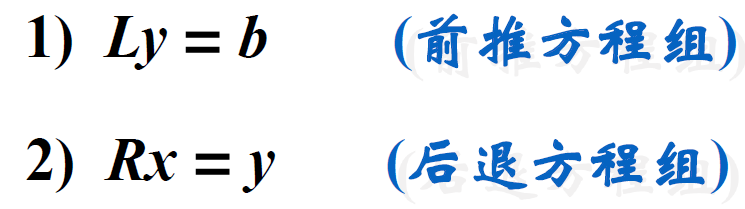这2个方程很容易解决，首先因为

L

\mathbf L

是单位下三角矩阵，则方程

L

y

=

b

\mathbf L \mathbf y=\mathbf b

可以变为如下：

[

1

l

21

1

⋮

⋱

⋱

l

n

1

⋯

l

m

n

−

1

1

]

[

y

1

y

2

⋮

y

n

]

=

[

b

1

b

2

⋮

b

n

]

\left[\begin{array}{cccc}{1} & {} & {} & {} \\ {l_{21}} & {1} & {} & {} \\ {\vdots} & {\ddots} & {\ddots} & {} \\ {l_{n 1}} & {\cdots} & {l_{m n-1}} & {1}\end{array}\right]\left[\begin{array}{c}{y_{1}} \\ {y_{2}} \\ {\vdots} \\ {y_{n}}\end{array}\right]=\left[\begin{array}{c}{b_{1}} \\ {b_{2}} \\ {\vdots} \\ {b_{n}}\end{array}\right]

其中：可以明显发现

y

1

=

b

1

y_1 = b_1

，然后可以推出

y

2

y_2

，以此类推！

y

1

⇒

y

2

⇒

⋯

⇒

y

n

y_{1} \Rightarrow y_{2} \Rightarrow \cdots \Rightarrow y_{n}

再由方程组

R

x

=

y

\mathbf R \mathbf x=\mathbf y

[

r

11

r

12

⋯

r

1

n

r

22

⋱

r

2

n

⋱

⋮

r

n

n

]

[

x

1

x

2

⋮

x

n

]

=

[

y

1

y

2

⋮

y

n

]

\left[\begin{array}{cccc}{r_{11}} & {r_{12}} & {\cdots} & {r_{1 n}} \\ {} & {r_{22}} & {\ddots} & {r_{2 n}} \\ {} & {} & {\ddots} & {\vdots} \\ {} & {} & {} & {r_{n n}}\end{array}\right]\left[\begin{array}{c}{x_{1}} \\ {x_{2}} \\ {\vdots} \\ {x_{n}}\end{array}\right]=\left[\begin{array}{c}{y_{1}} \\ {y_{2}} \\ {\vdots} \\ {y_{n}}\end{array}\right]

其中

x

n

=

r

n

n

x_n=r_{nn}

，最终我们可以得到：

x

n

⇒

x

n

−

1

⇒

⋯

⇒

x

1

x_{n} \Rightarrow x_{n-1} \Rightarrow \cdots \Rightarrow x_{1}

所以三角分解的一个很重要的应用就是用来解非齐次线性方程组，其可以简化运算。
1.4. 三角分解的充要条件：定理1
定理1：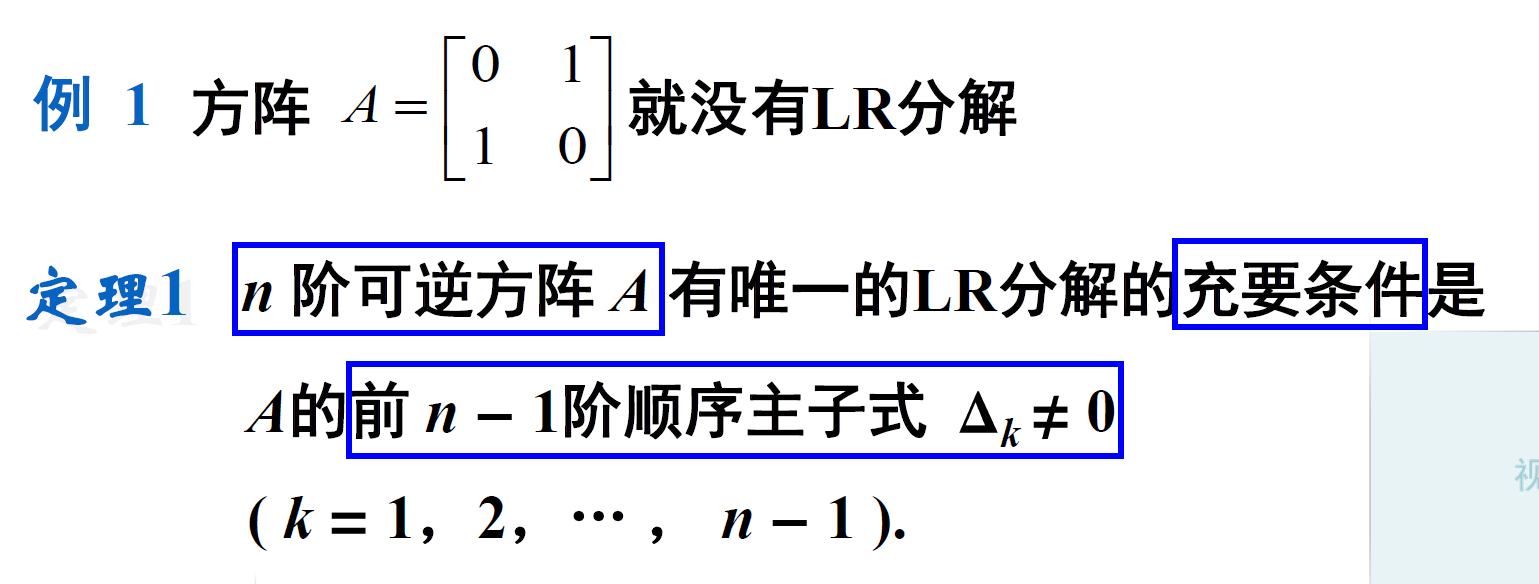先证明充要性：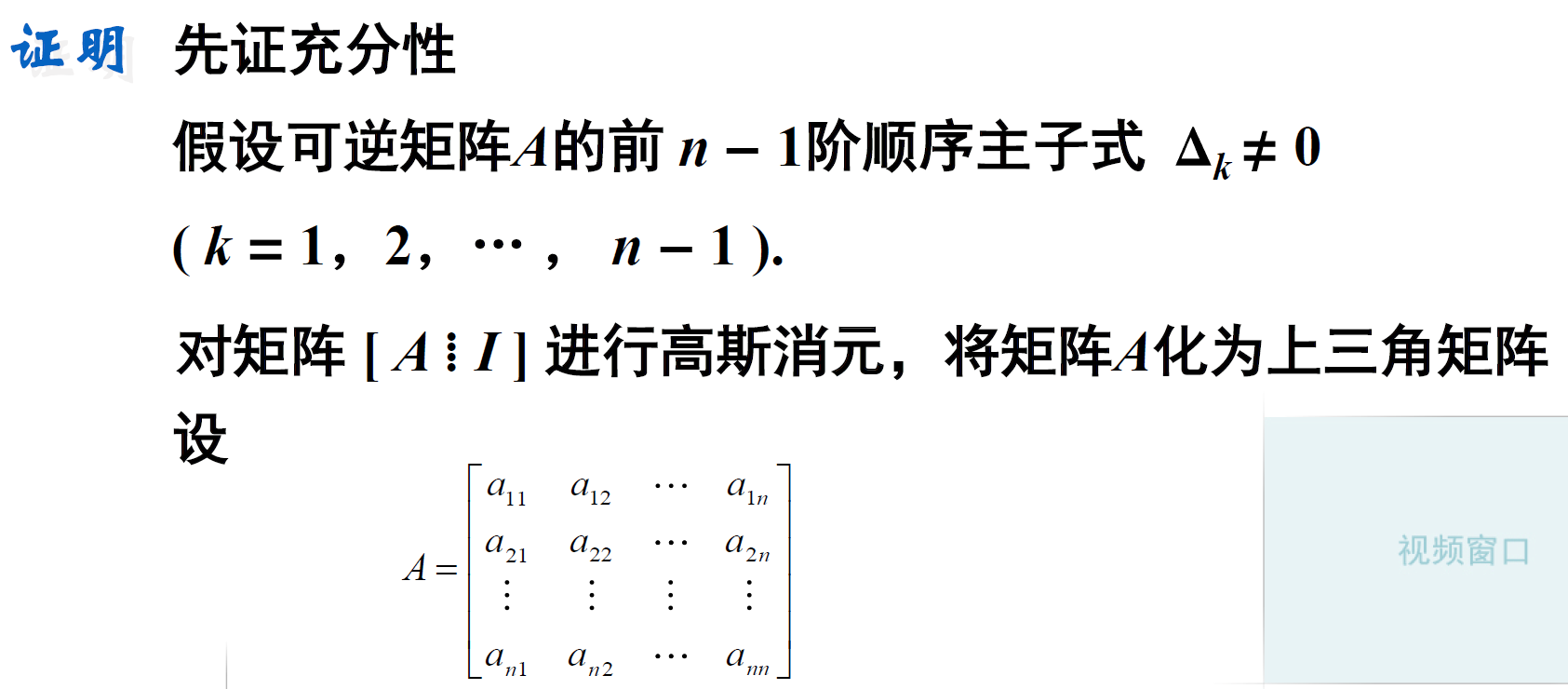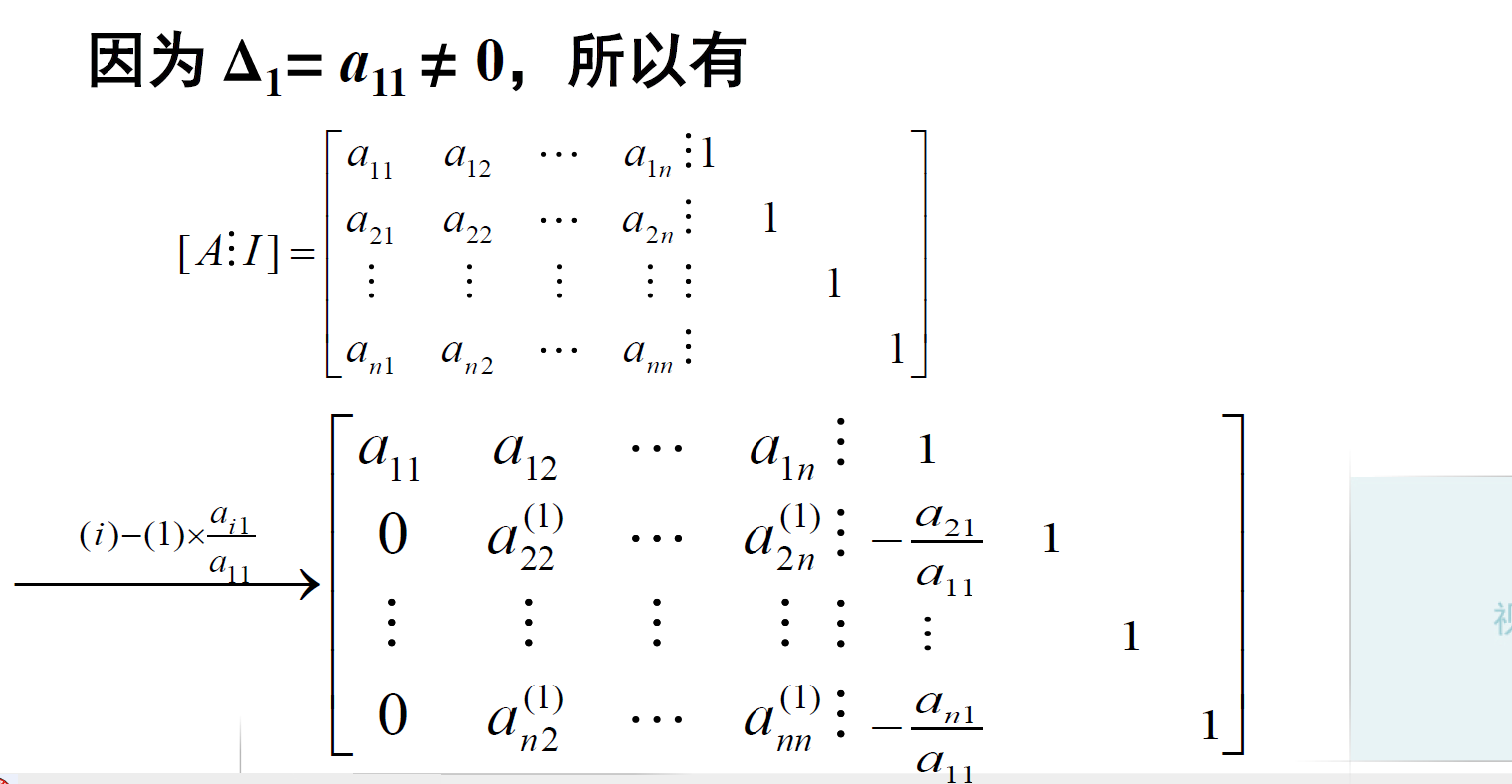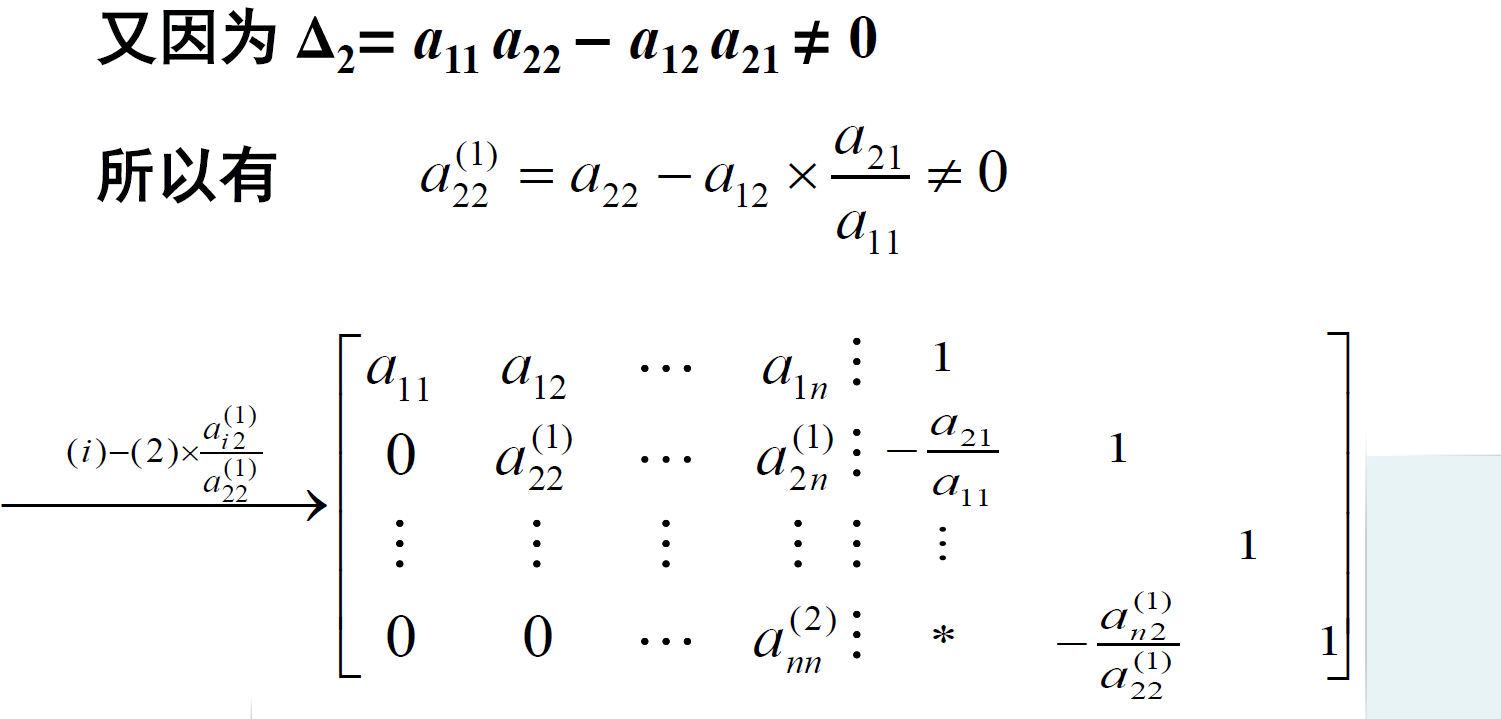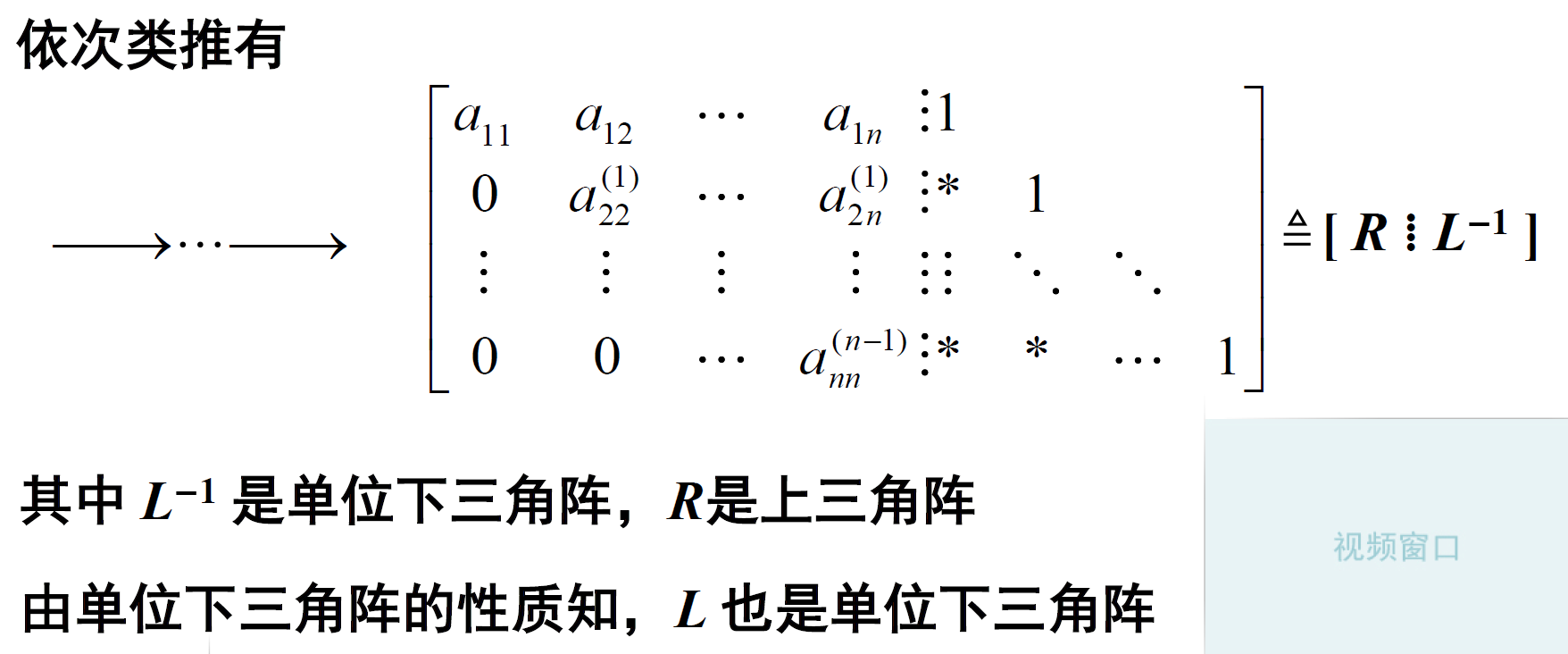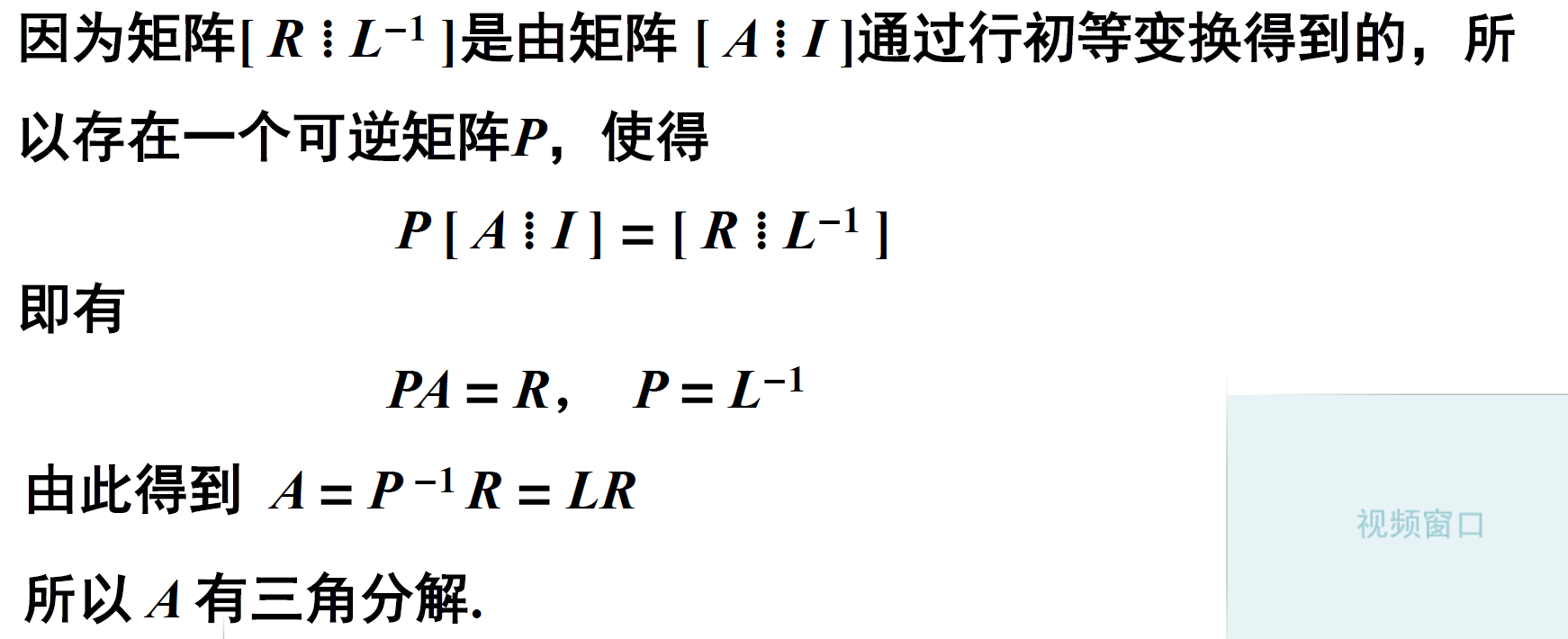下面证明唯一性：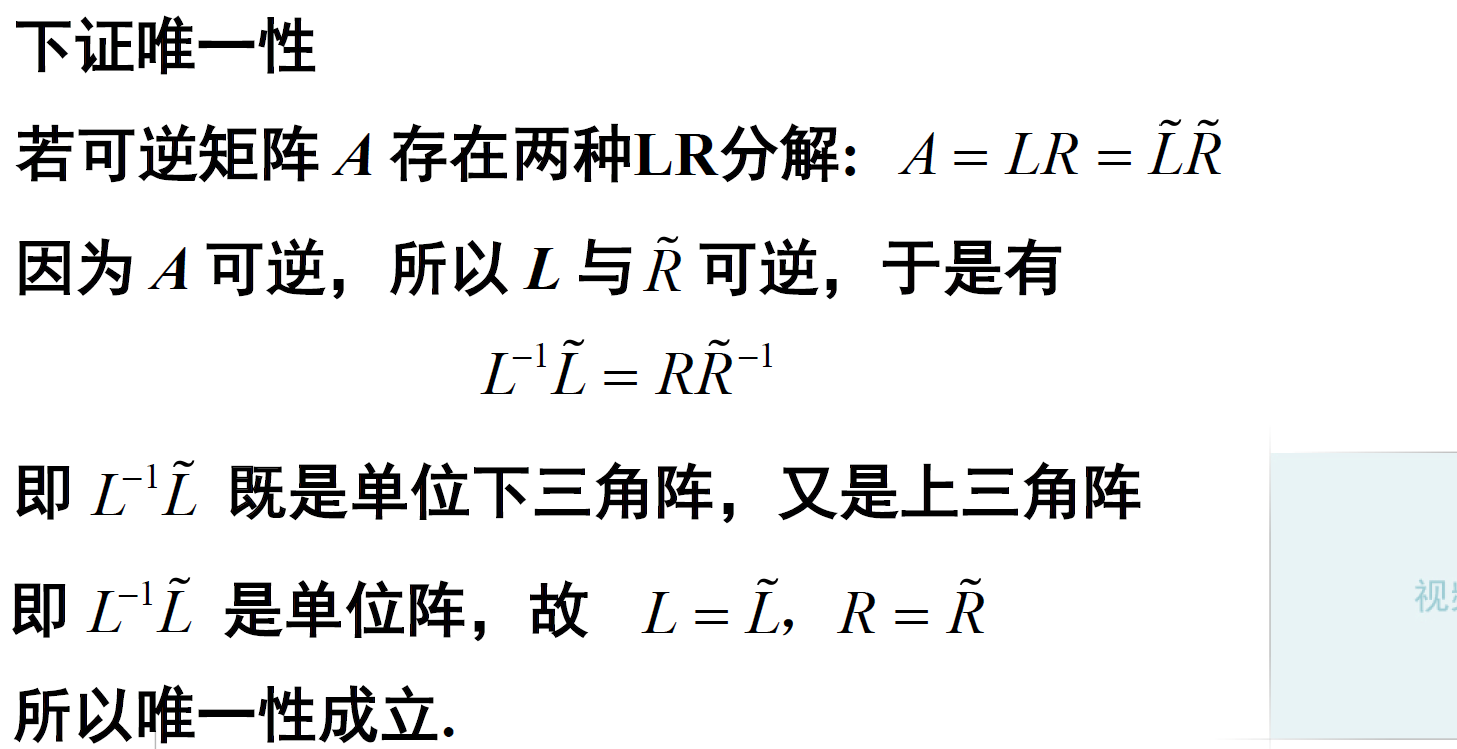下面证明必要性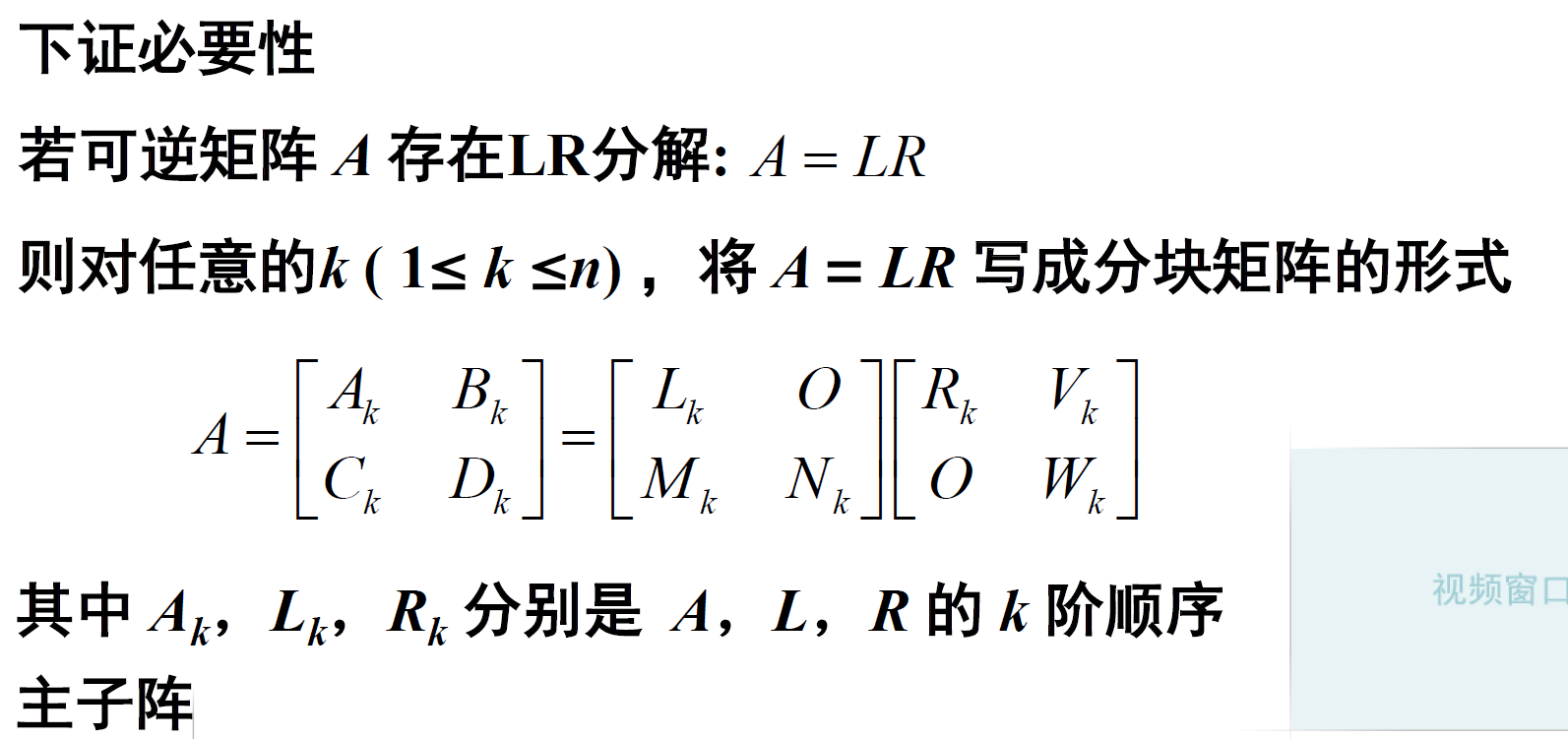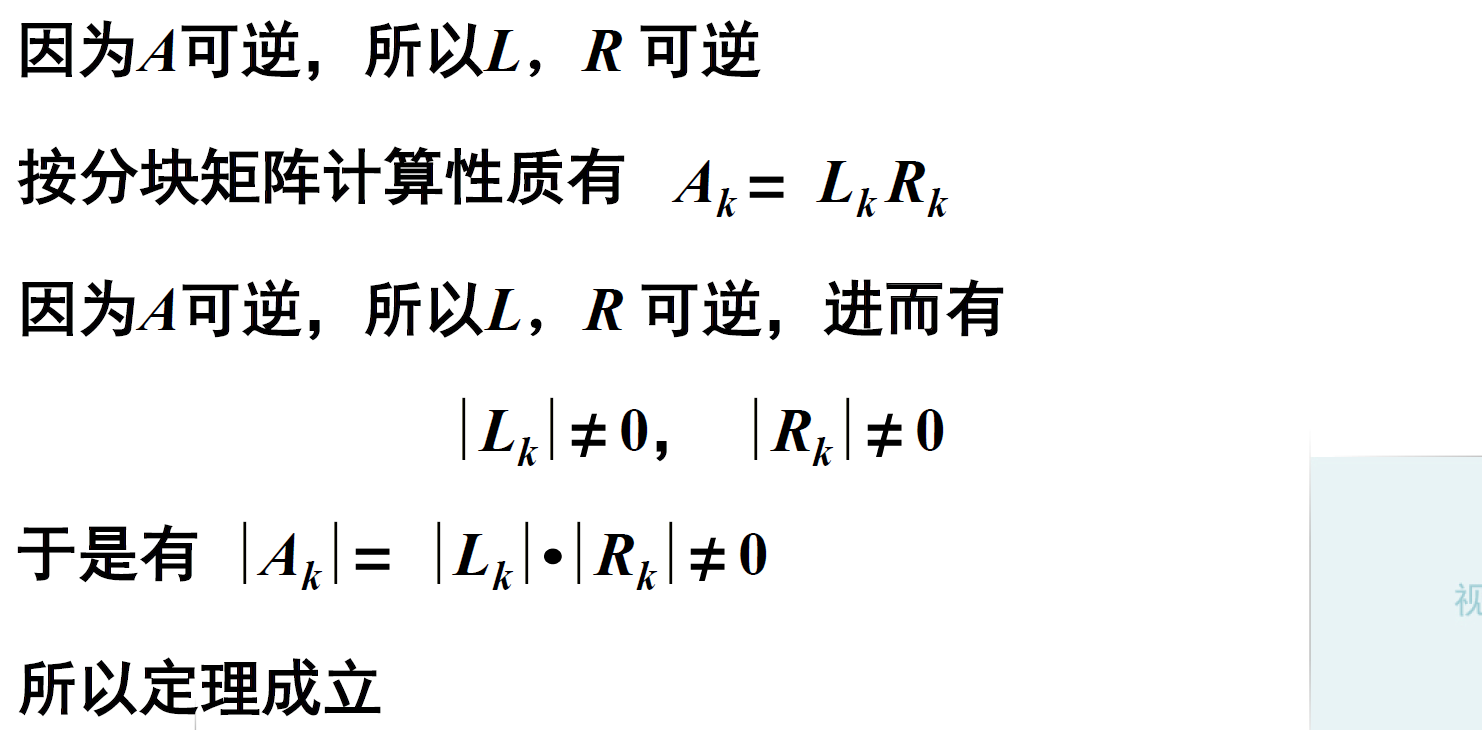二. 三角分解(LR分解)的求解
2.1. 三角分解的方法之高斯消元法
下面的例子1：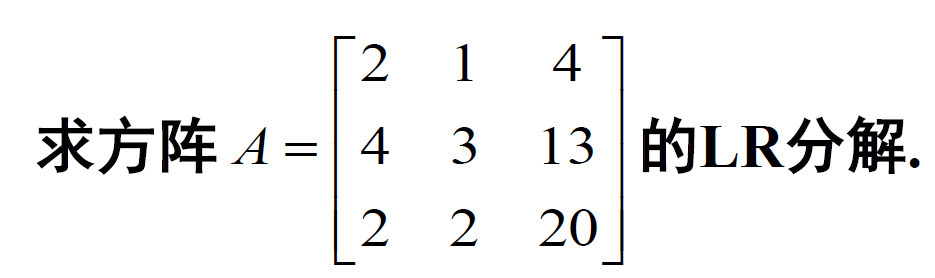这里也是按照前一小节中证明充分性的方法。首先我们判断一下 n-1 阶顺序主子式是否有0：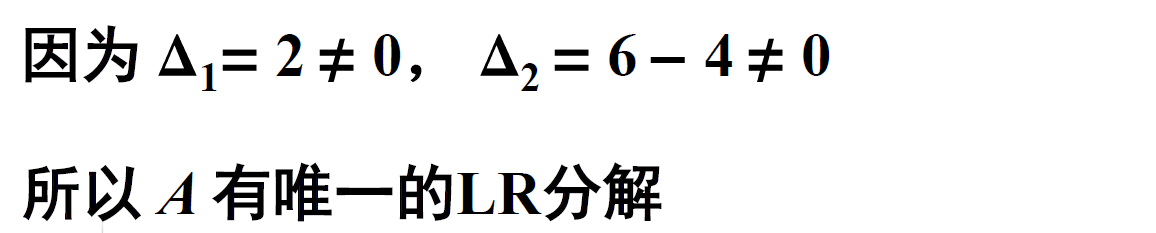下面要用到高斯消元法，再系数矩阵的右边添加一个单位矩阵变成一个增广矩阵：参考链接：增广矩阵！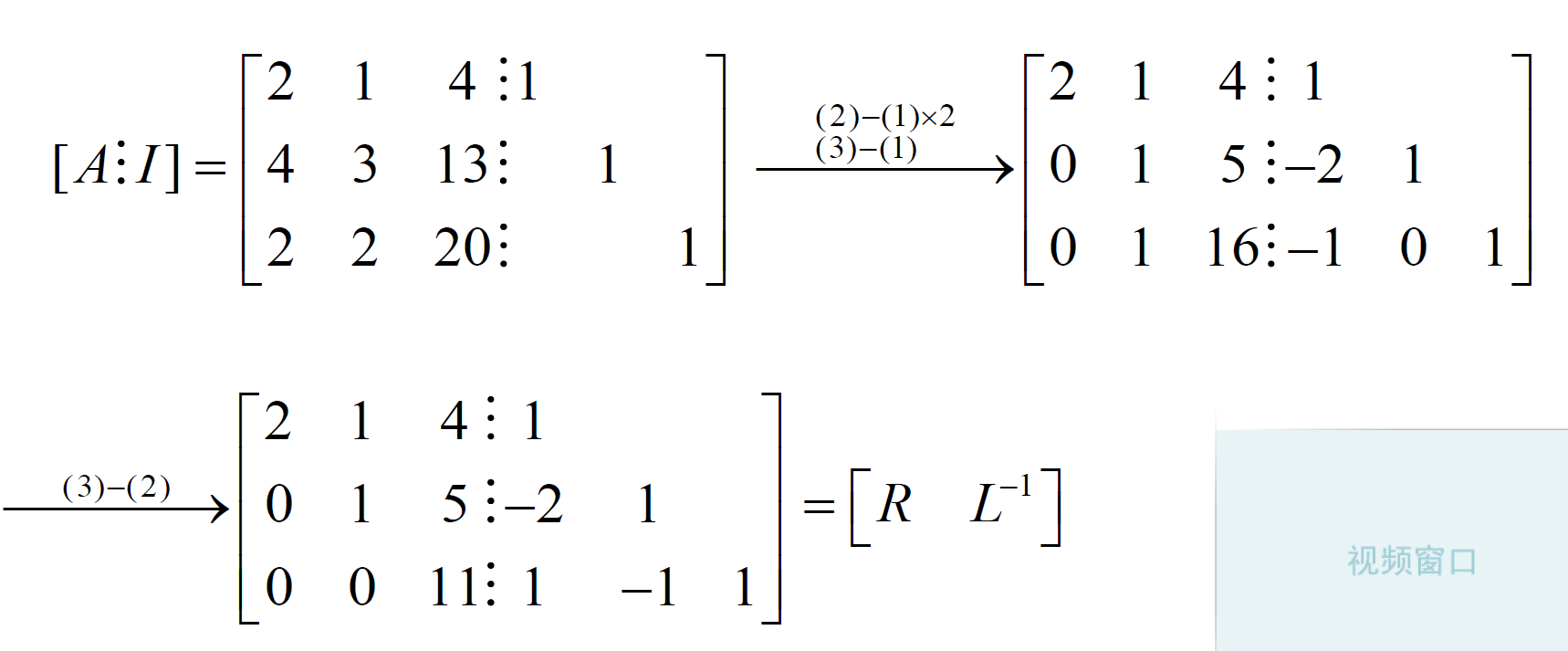这里我们已经把

R

\mathbf R

算出来了就是前面的上三角矩阵，后面的是

L

−

1

\mathbf L^{-1}

，我们只需要对

L

−

1

\mathbf L^{-1}

再求逆就可以得到了

L

\mathbf L

；这就是第一种方法高斯消元法求解三角分解，其中有求逆相对麻烦一些。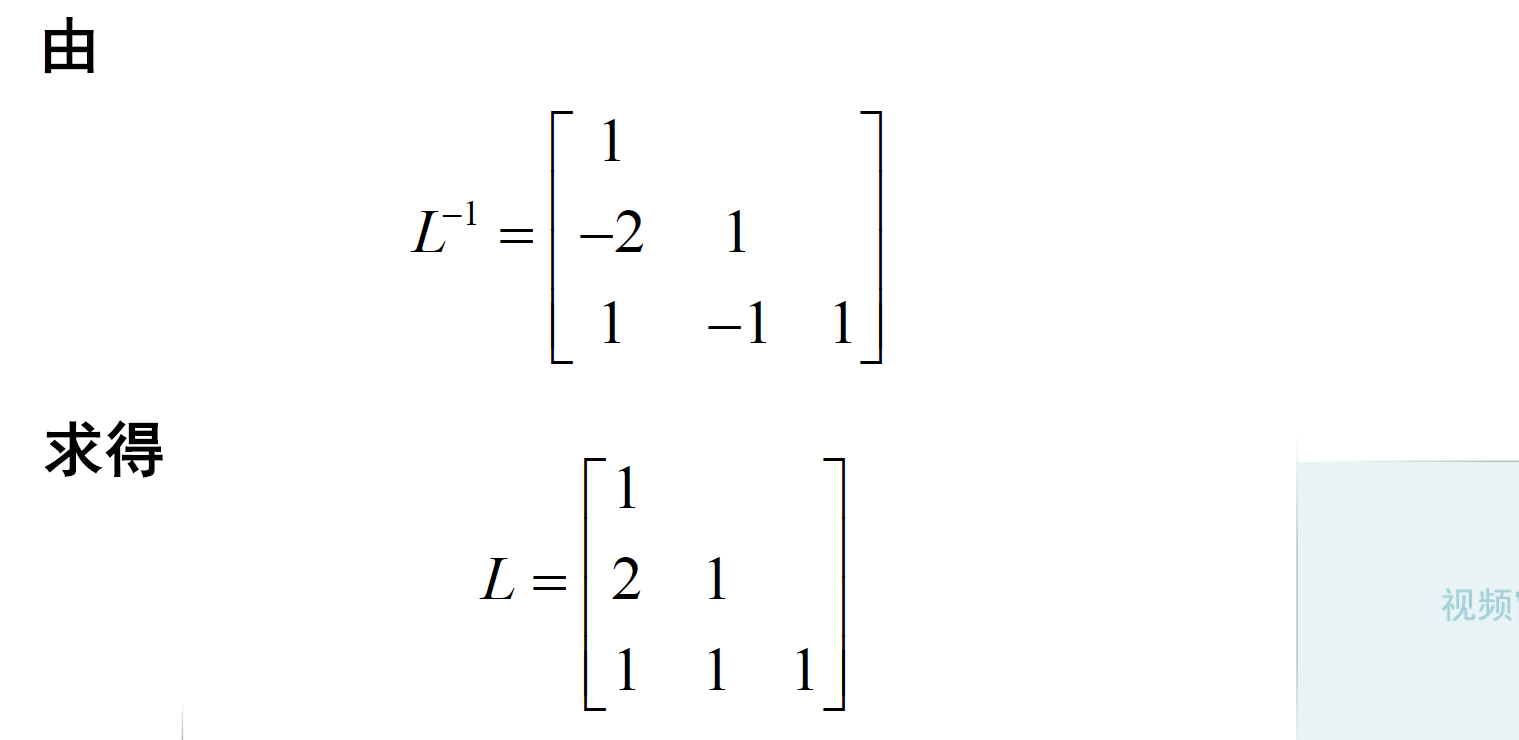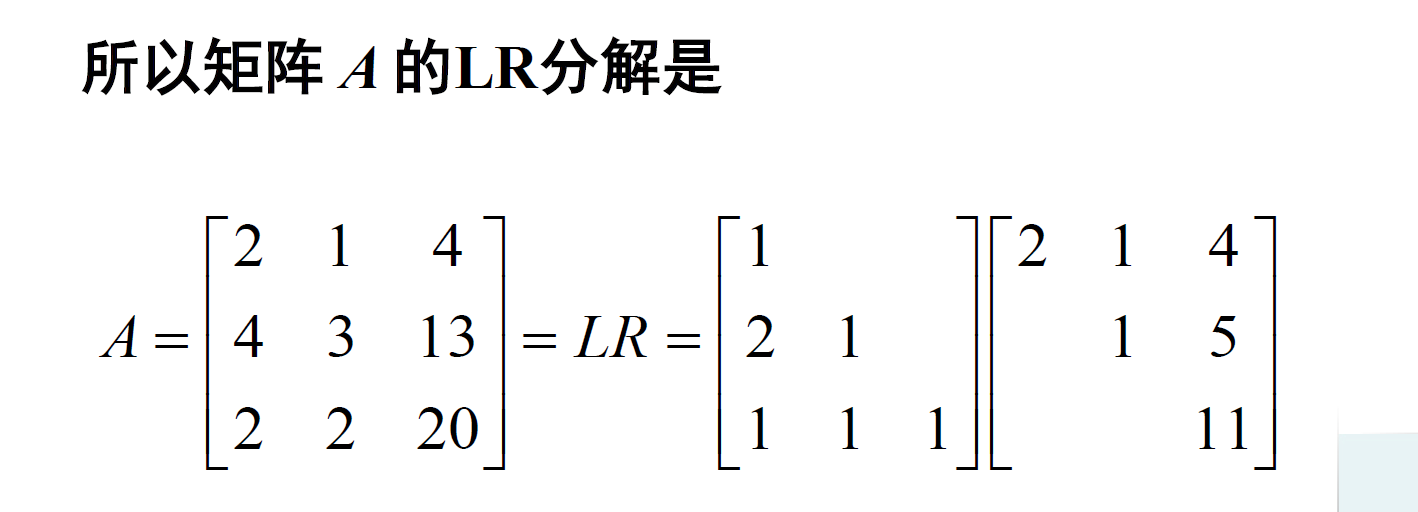下面的例子2：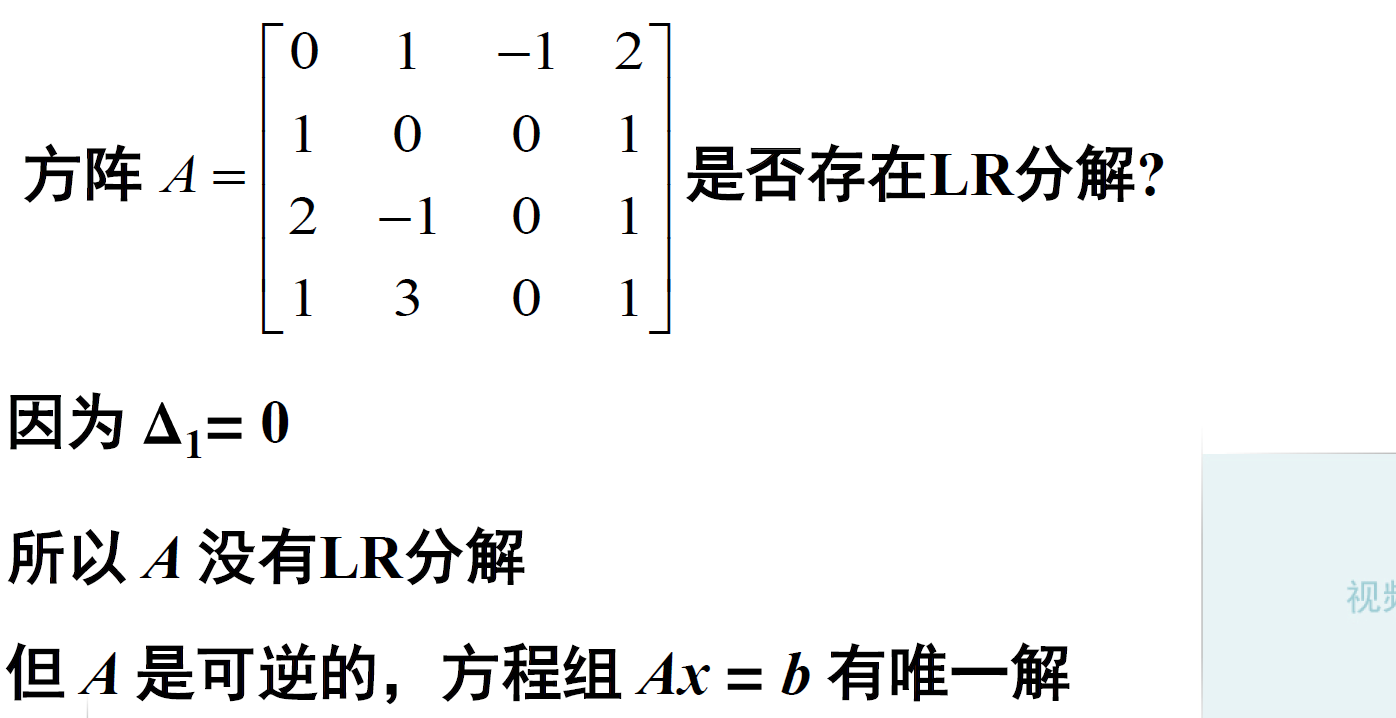不存在LR分解，但是刚才我们介绍过三角分解主要作用就是用来求解线性方程的。解方程的时候只要这个矩阵是可逆的，肯定存在解。那么这个矩阵是可逆的，因此这个方程是有唯一解的。如果我们想用三角分解求解这个方程，怎么办呢？我们发现只要把这个矩阵的第一行和第二行交换一下，矩阵

A

\mathbf A

变为

A

~

\mathbf {\tilde{A}}

，这个矩阵是满足三角分解的条件的。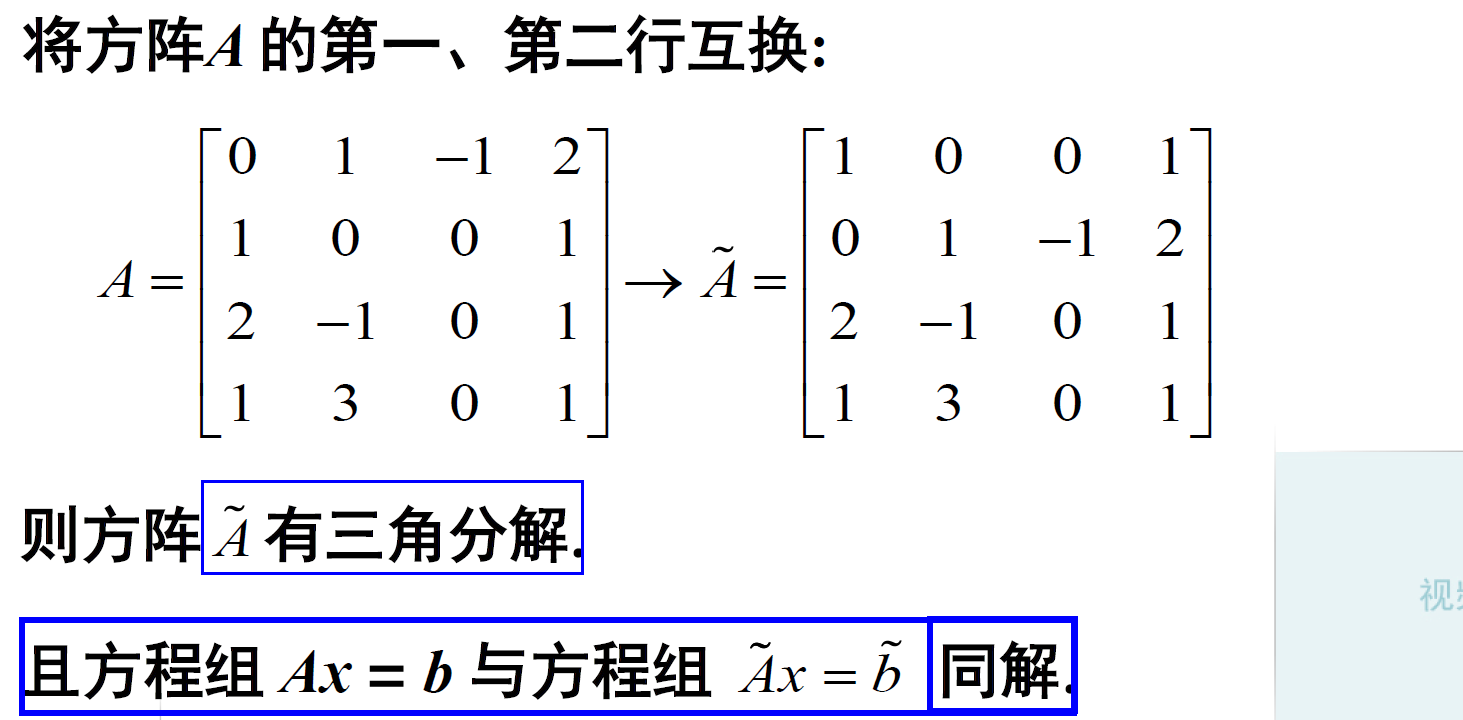那么问题来了？方阵

A

\mathbf A

和

A

~

\mathbf {\tilde{A}}

有什么关系呢？注意： 置换矩阵就是单位阵置换行以后的矩阵。

2.2. 带行交换的LR分解：定理2
定理2：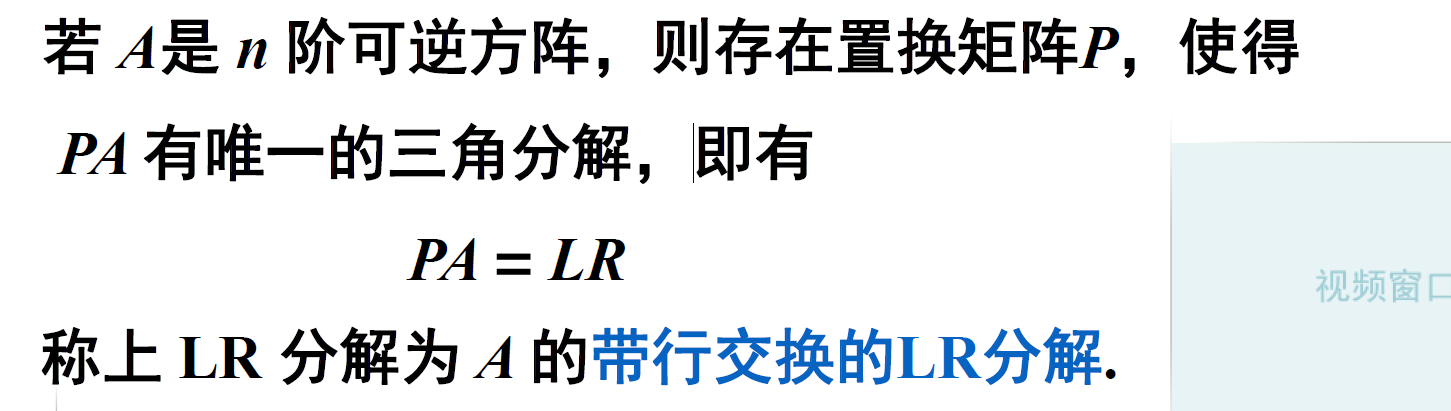下面的例子1：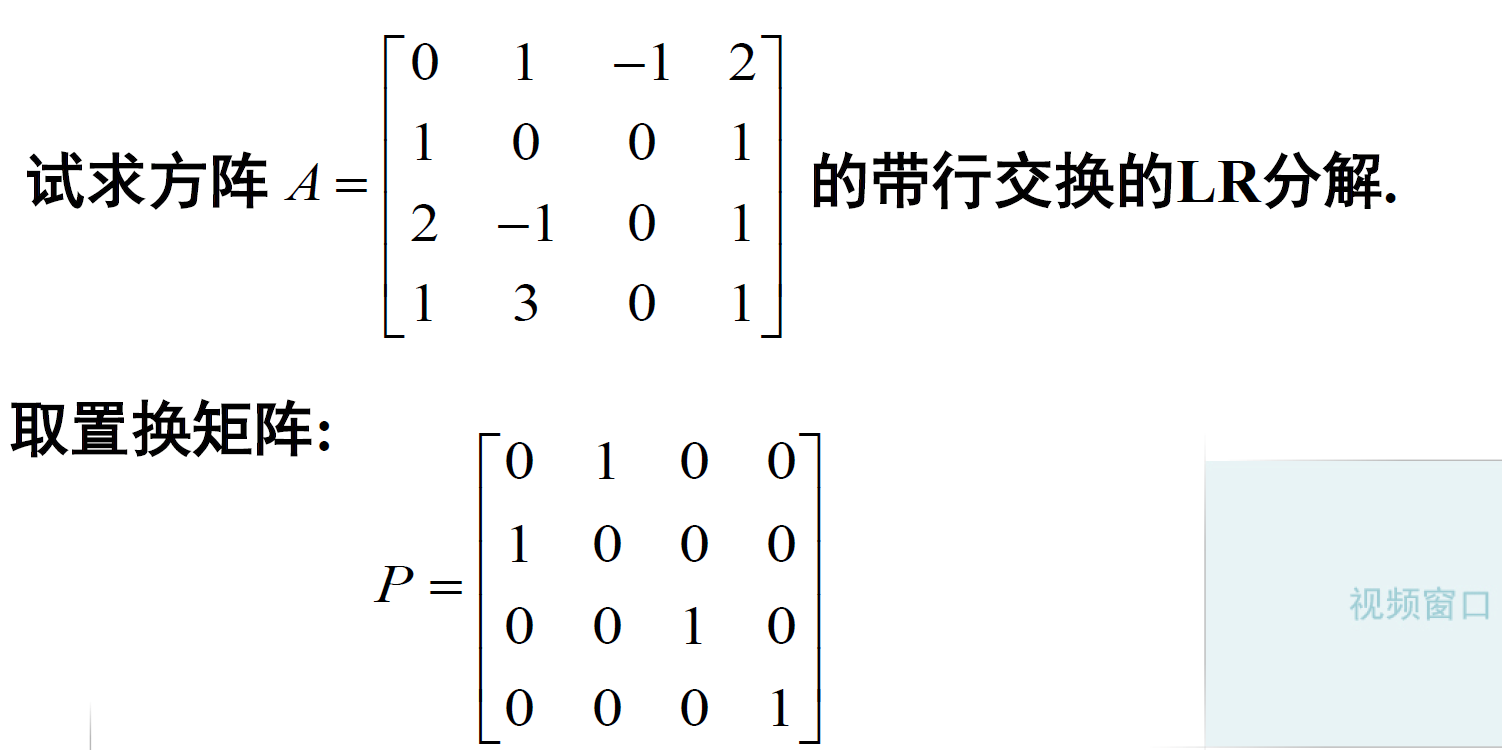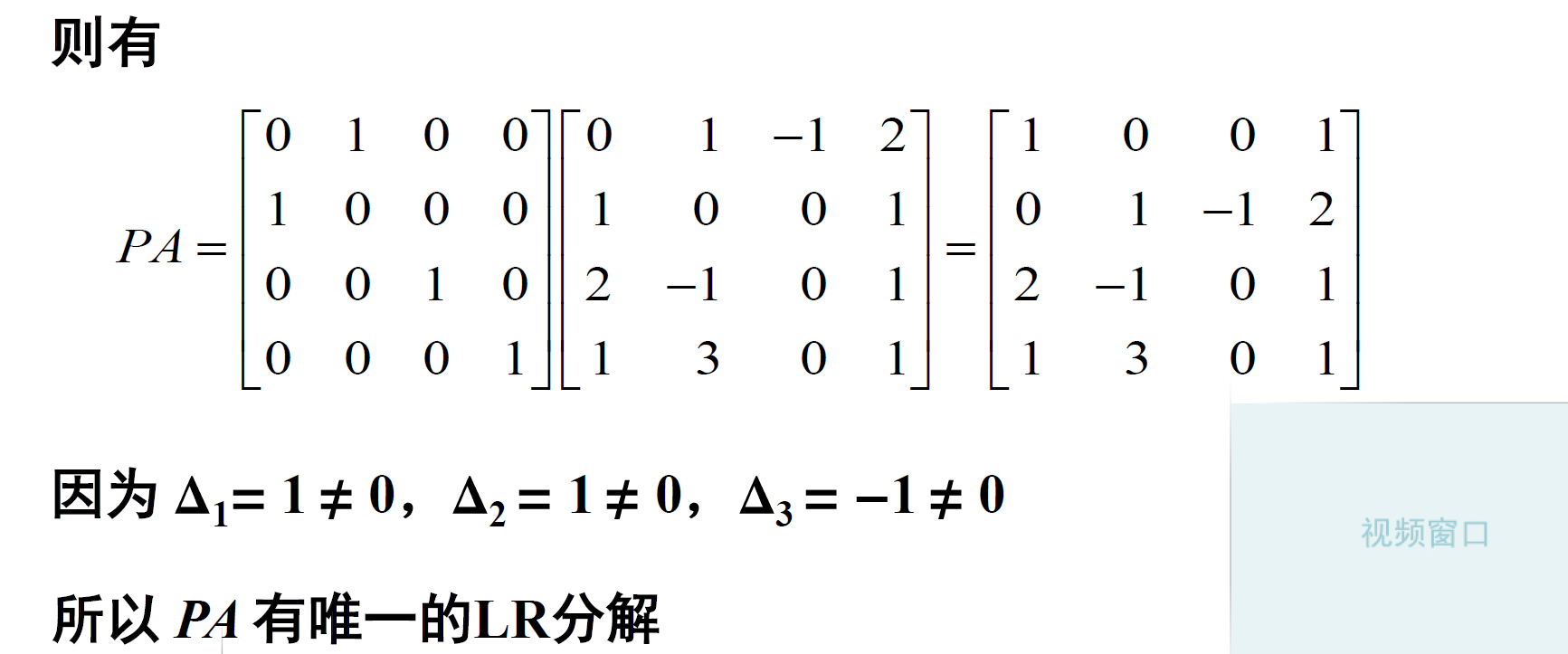下面利用高斯消元法求解：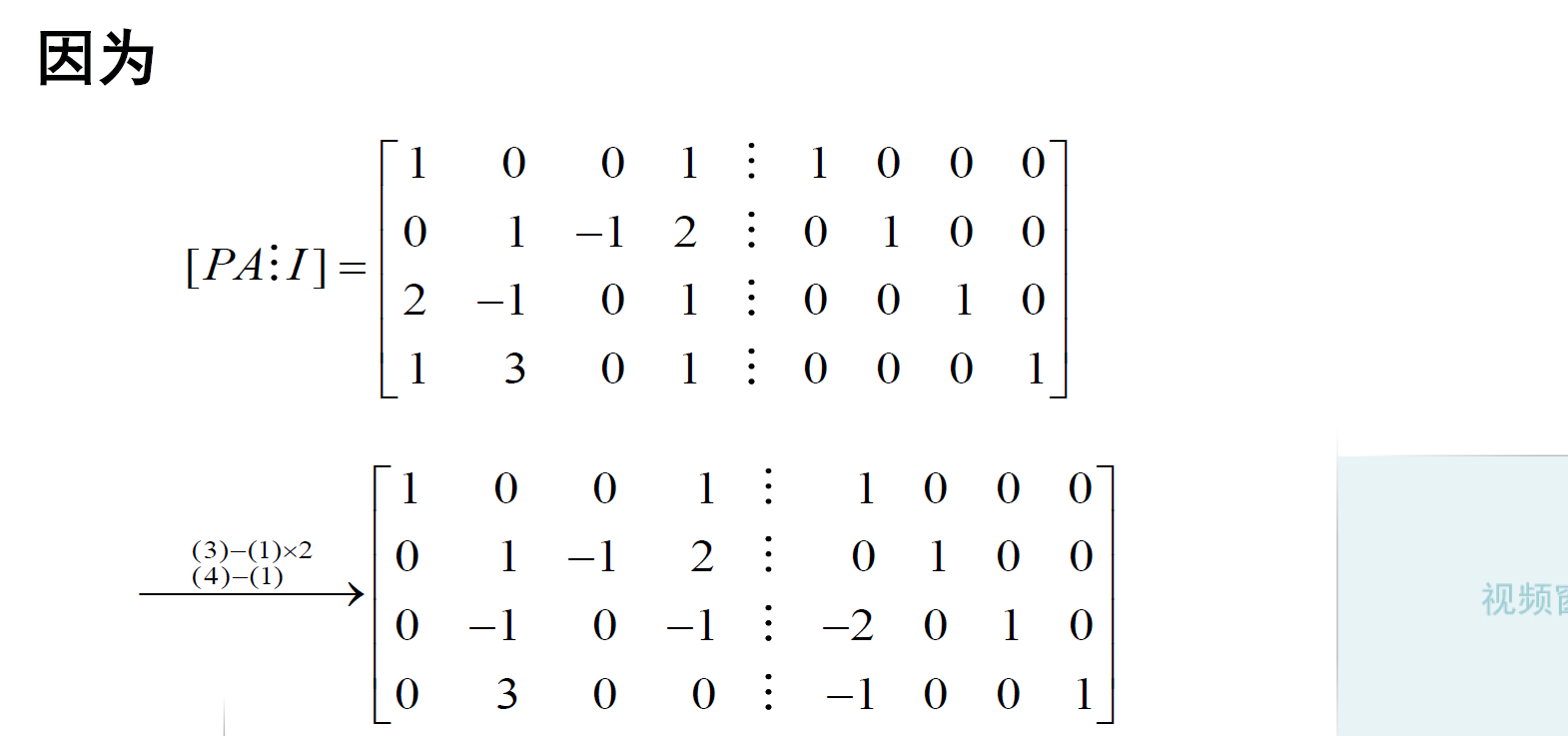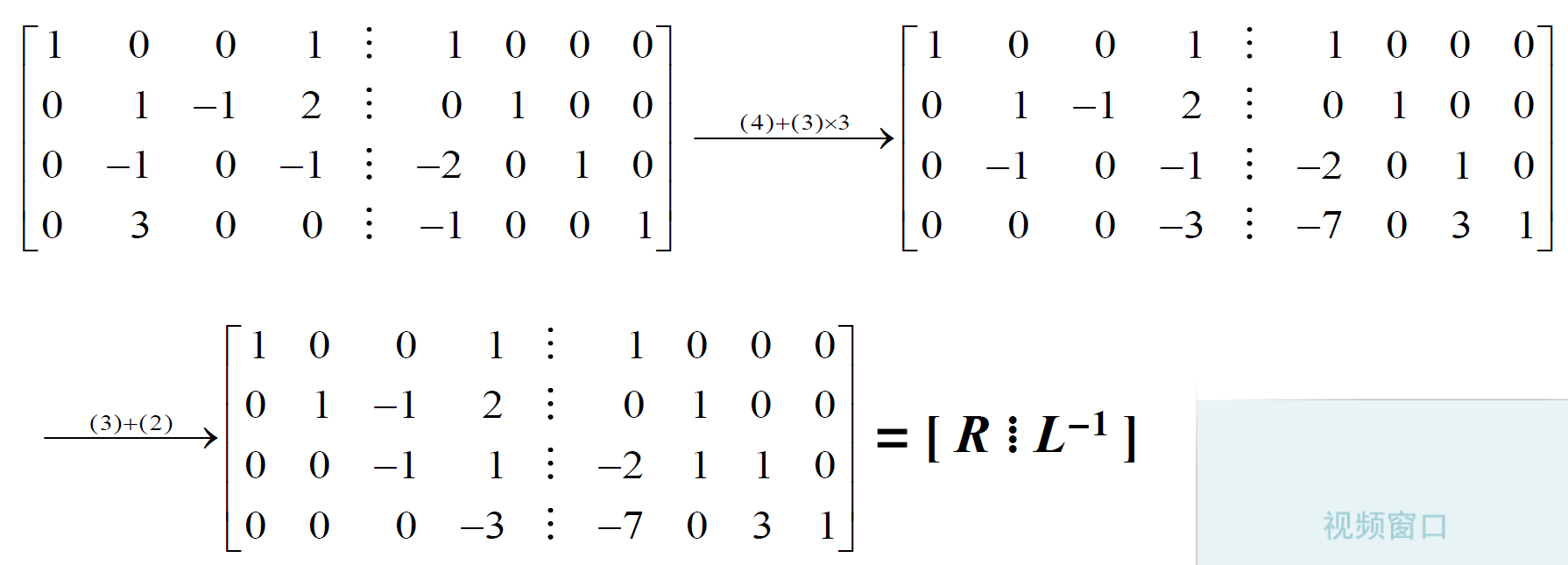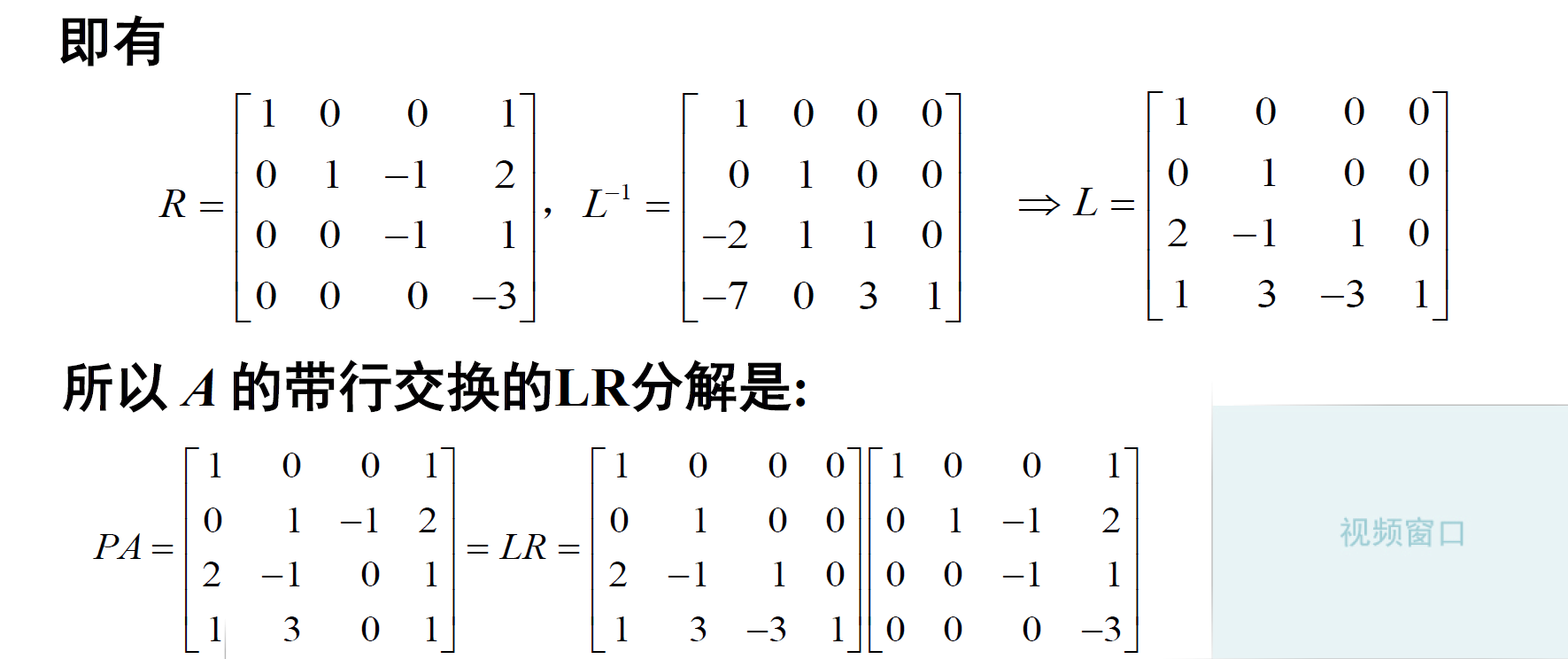2.3. 三角分解的方法之待定系数法
通过前面的求解我们发现高斯消元法有一个问题：就是我们求解完得到的有个

L

−

1

\mathbf L^{-1}

，我们需要转变求解其逆矩阵得到

L

\mathbf L

，这样求解比较麻烦，所以编程实现也比较麻烦！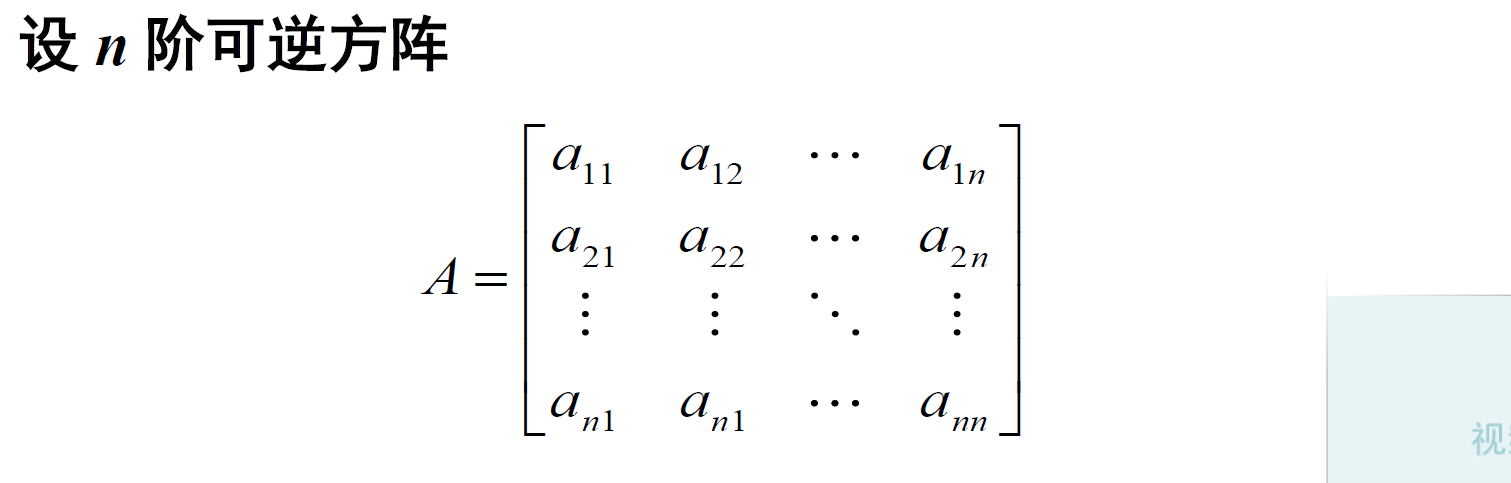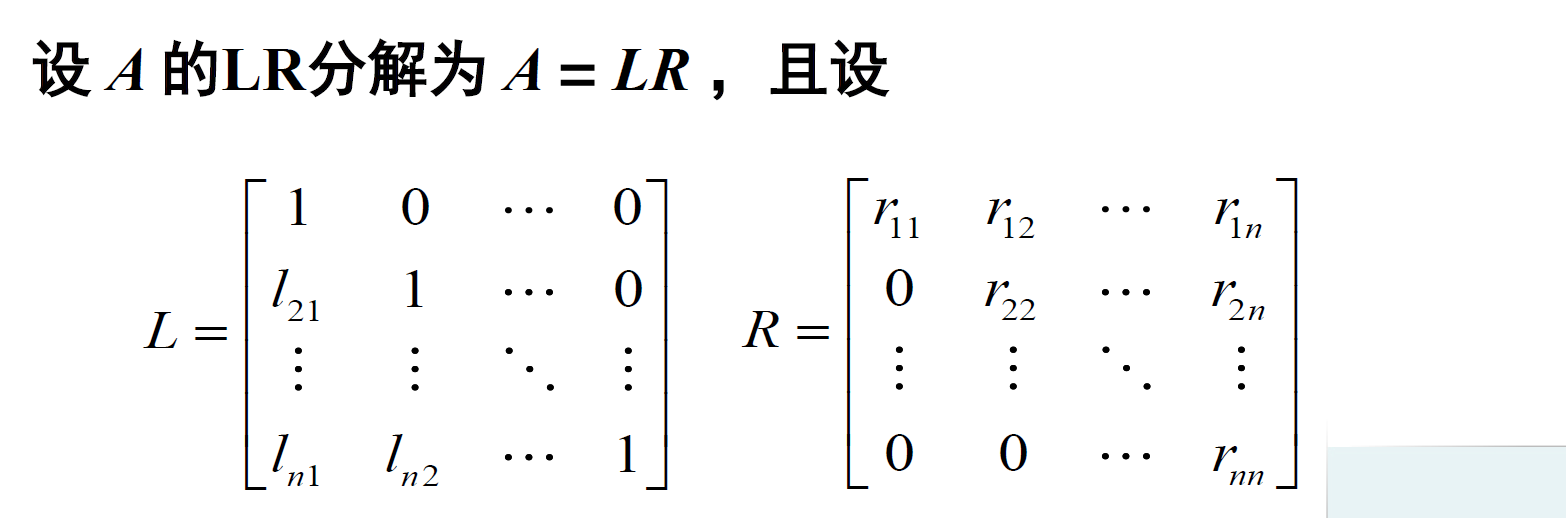由矩阵的乘法可以知道，

L

R

\mathbf L \mathbf R

相乘的结果和

A

\mathbf A

对应位置相等。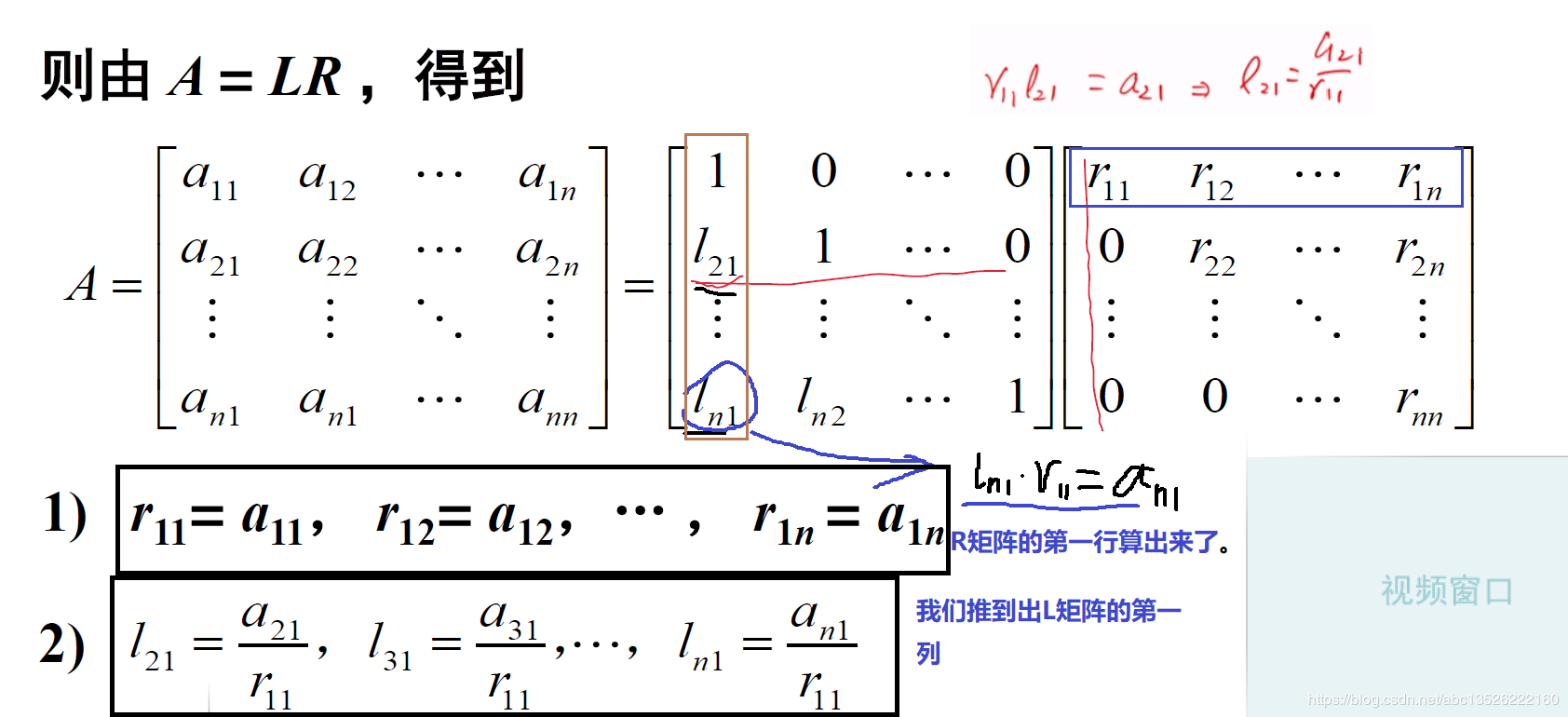我们是如何推导的呢？先算

R

\mathbf R

的第一行，它和

A

\mathbf A

的第一行是一样的，算完之后我们就可以算出来

L

\mathbf L

的第一列。剩下我们再计算

R

\mathbf R

的第二行，用算

L

\mathbf L

的第二行乘以

R

\mathbf R

的第二列，得到如下的3)和4)；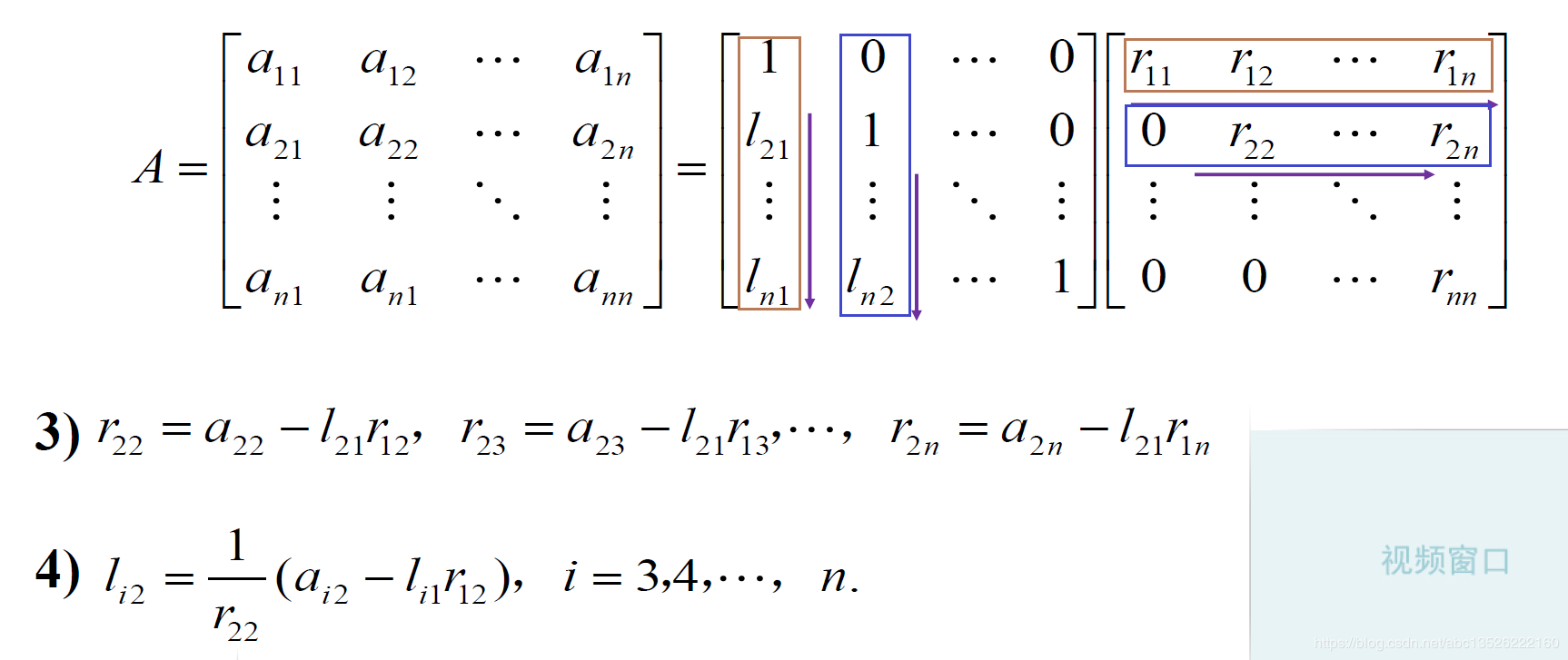由此得到一般的公式(这个公式看着复杂，这个公式记不住没关系，我们知道先计算

R

\mathbf R

的第一行，再计算

L

\mathbf L

的第一列，依次进行下去(记住这个顺序)… )：

r

k

j

=

a

k

j

−

∑

i

=

1

k

−

1

l

k

i

r

i

j

,

j

=

k

,

k

+

1

,

⋯

,

n

r_{k j}=a_{k j}-\sum_{i=1}^{k-1} l_{k i} r_{i j}, \quad j=k, \quad k+1, \cdots, n

l

i

k

=

1

r

k

k

(

a

i

k

−

∑

m

=

1

k

−

1

l

i

m

r

m

k

)

,

i

=

k

+

1

,

⋯

,

n

l_{i k}=\frac{1}{r_{k k}}\left(a_{i k}-\sum_{m=1}^{k-1} l_{i m} r_{m k}\right), \quad i=k+1, \cdots, n

下面的例子1：
这里我们直接用待定系数法，不用高斯消元法，当然可以用这个。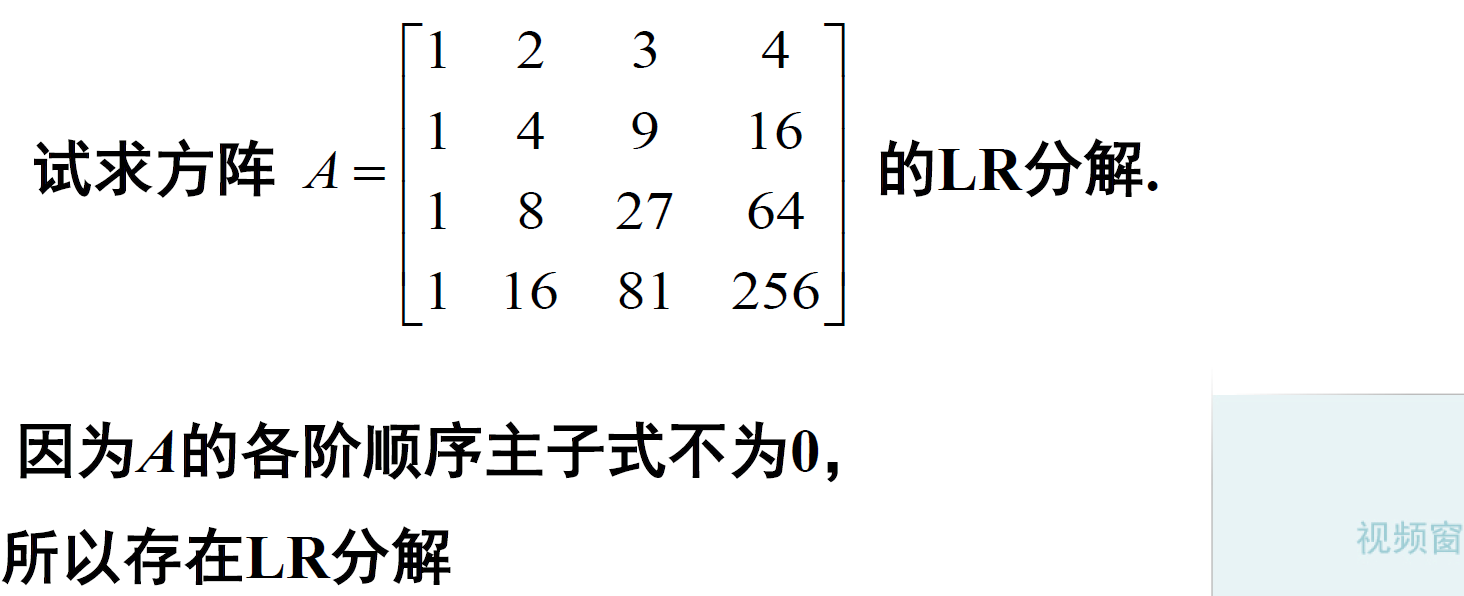我们首先计算

R

\mathbf R

的第一行，我们刚才知道

R

\mathbf R

的第一行和

A

\mathbf A

的第一行是一样的。 再算

L

\mathbf L

的第一列，依次进行下去。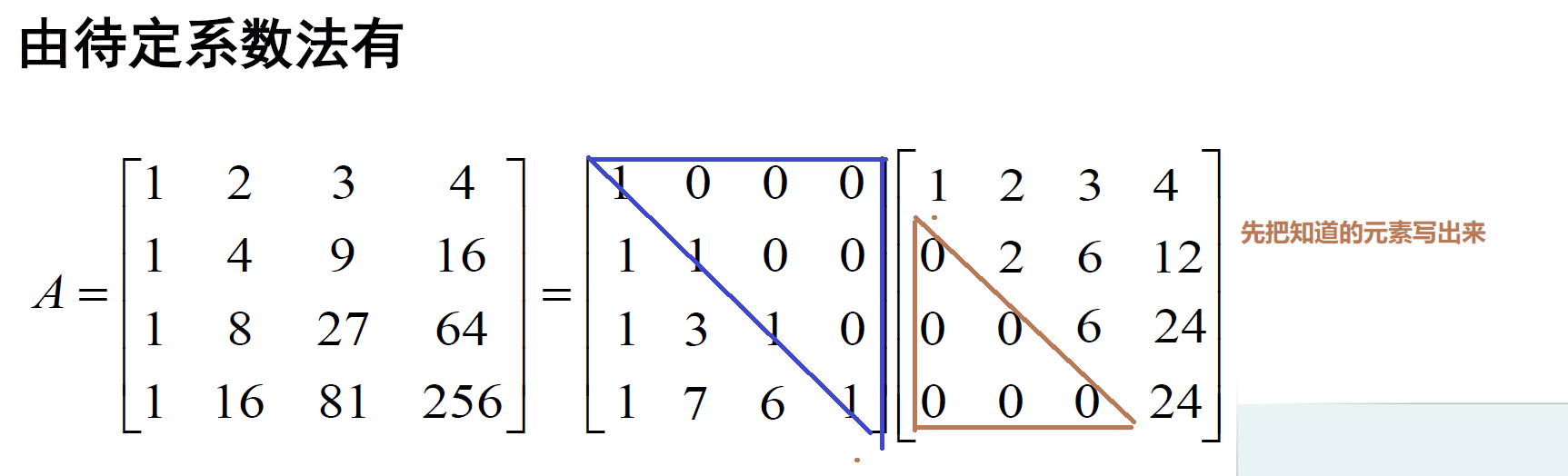三. 平方根分解(Cholesky分解)
下面我们介绍另外一种分解叫做平方根分解，首先介绍一下预备知识LDR分解。
3.1. LDR分解的定义(预备知识)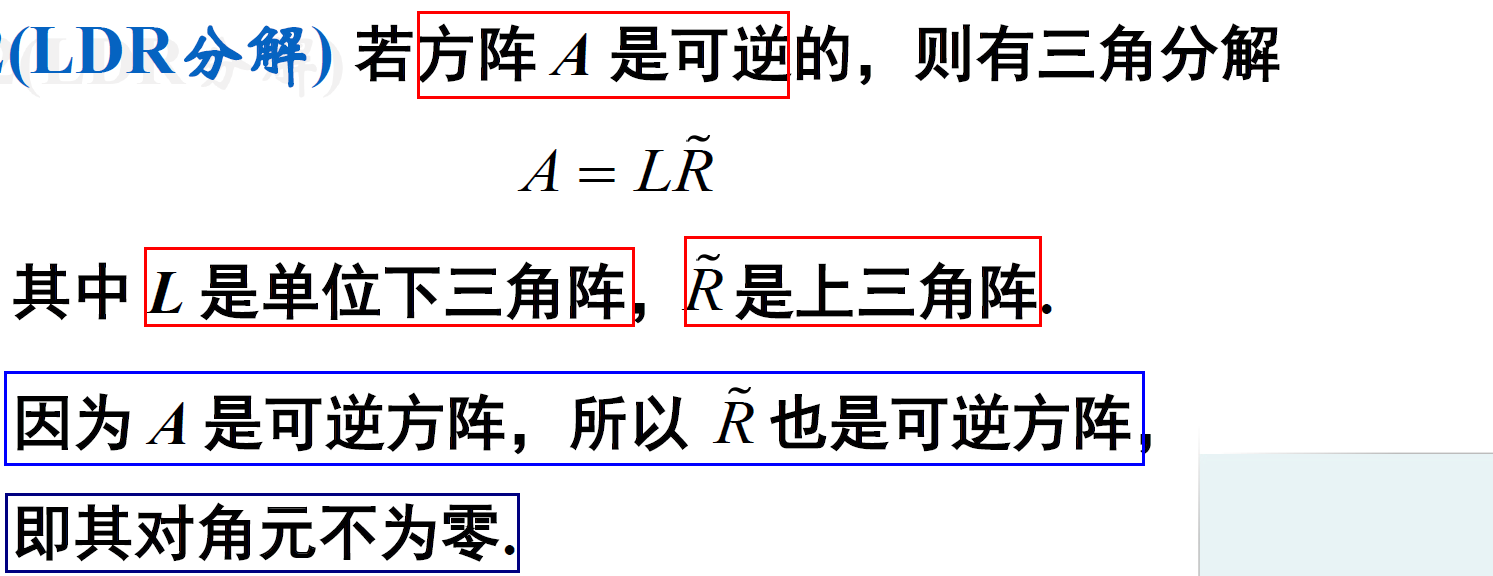这样得到的矩阵分解就是比较对称的了，所以怎么求LDR分解其实很简单：首先求解

A

\mathbf A

的三角分解(LR分解)

A

=

L

R

\mathbf A=\mathbf L \mathbf R

；然后把

R

\mathbf R

的对角元素取出来组成一个对角阵，然后

m

a

t

h

b

f

R

mathbf R

的每一行除以相应的对角元素。下面的例子1：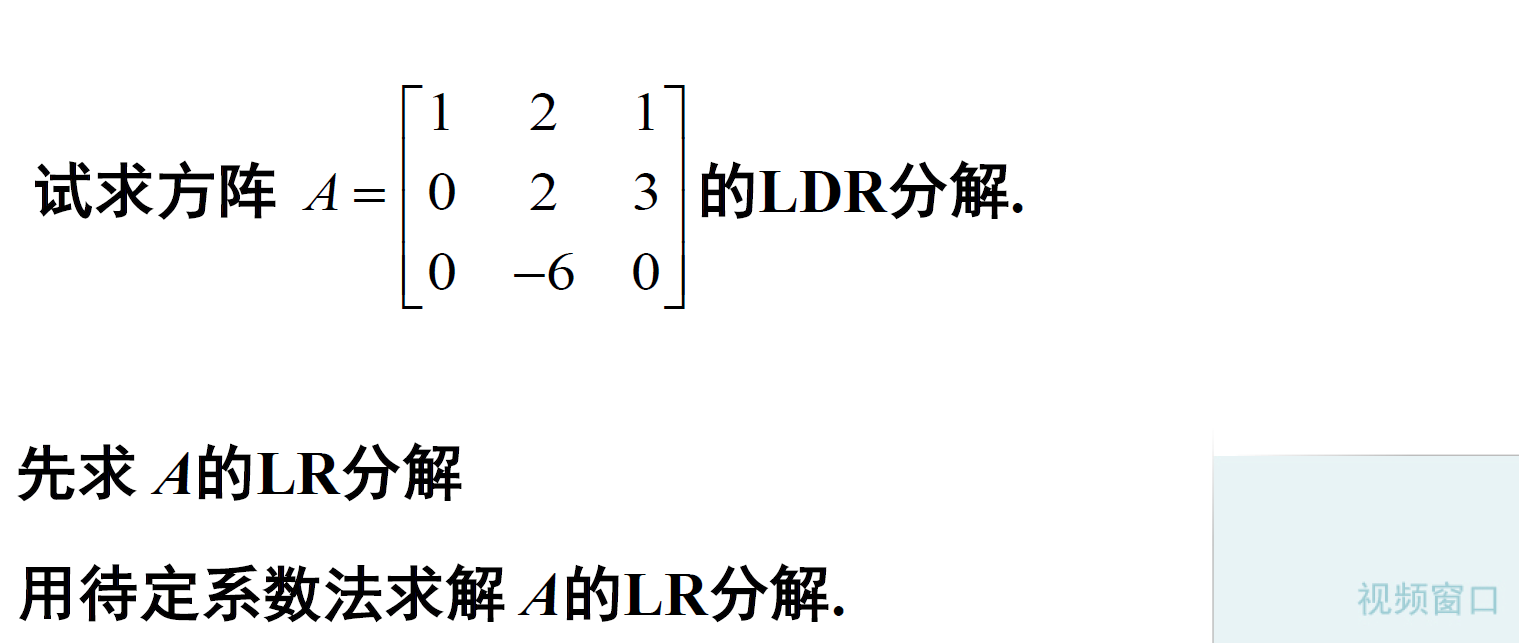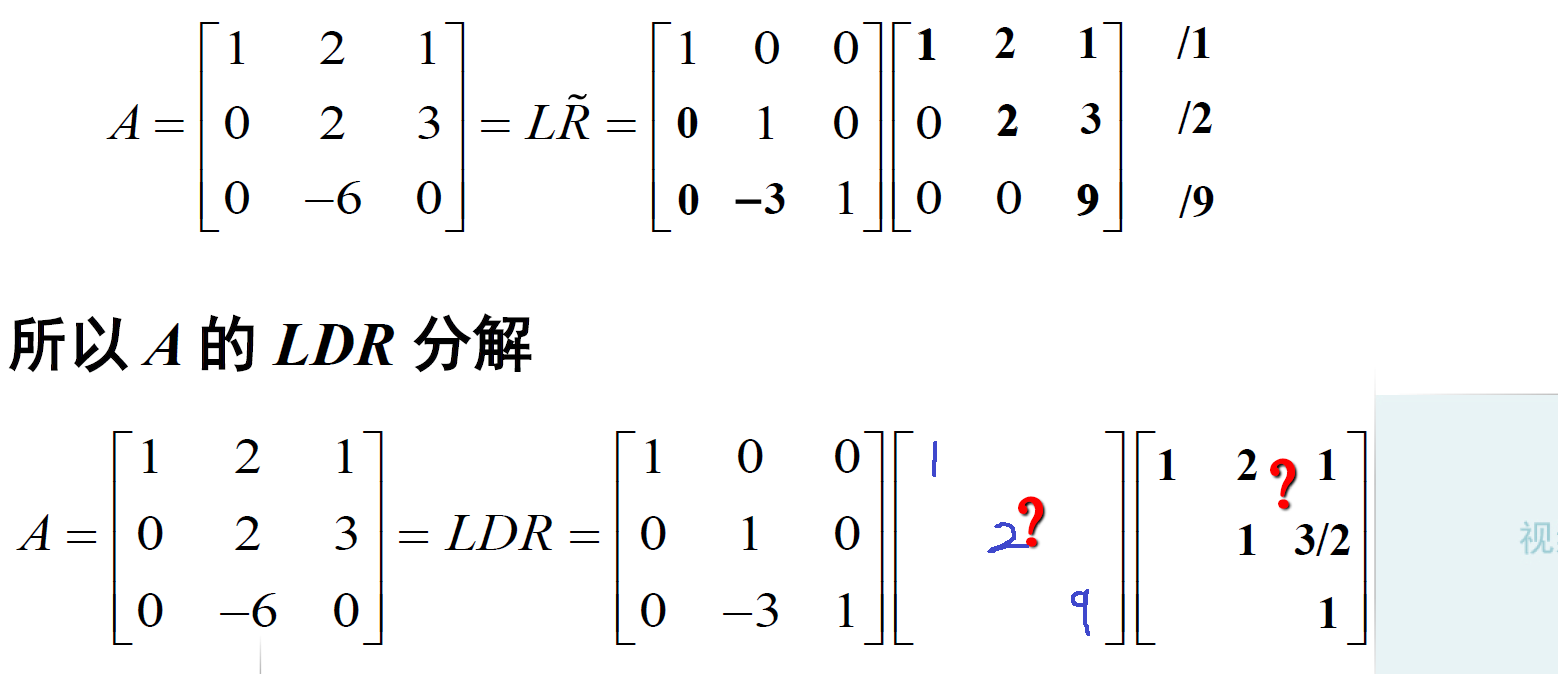3.2. 平方根分解(Cholesky分解)
如何矩阵

A

\mathbf A

是对称矩阵我们会得到什么结果？如果矩阵

A

\mathbf A

又是一个正定矩阵我们又会得到什么结论？这就是我们引出的平方根分解
正定矩阵

A

\mathbf A

分解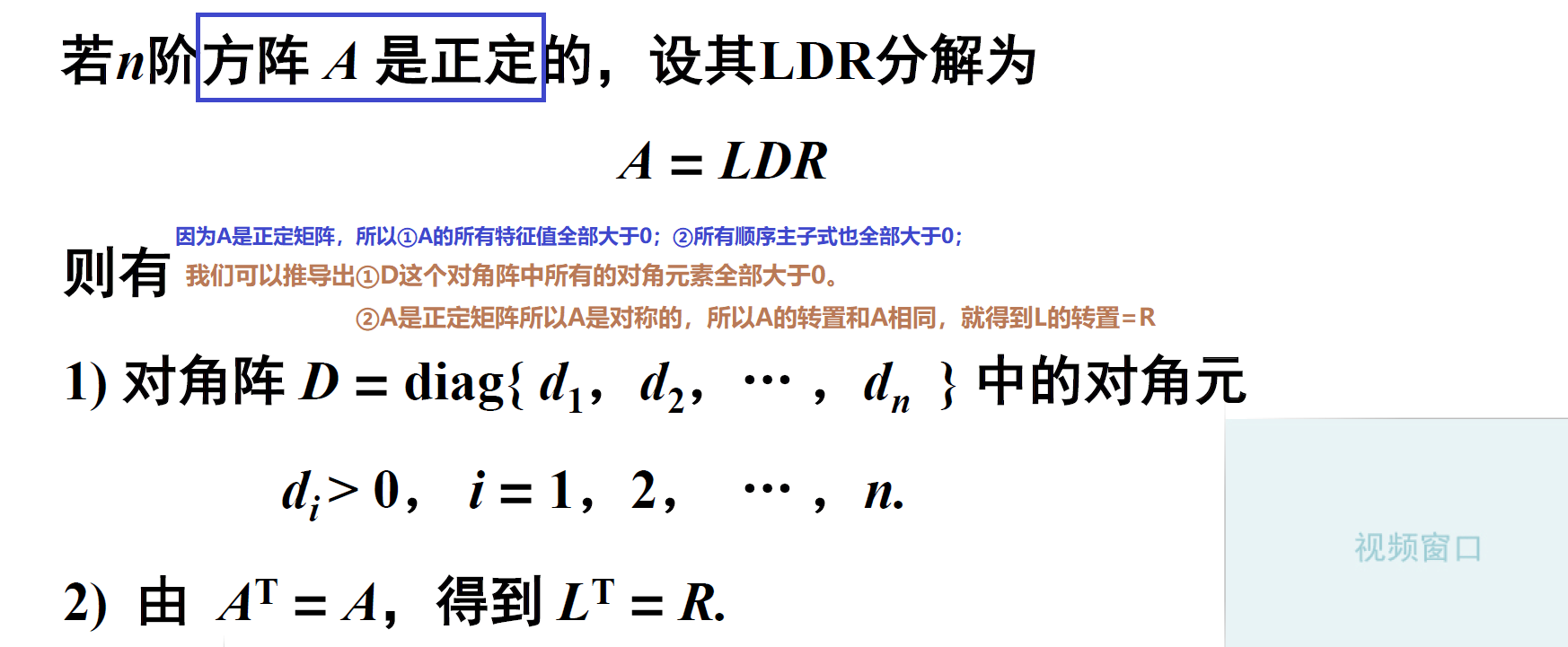注意： 为什么可以得到

L

T

=

R

\mathbf {L}^{\mathbf{T}}=\mathbf {R}

A

T

=

(

L

D

R

)

T

=

R

T

D

T

L

T

=

R

T

D

L

T

\begin{array}{l}{\mathbf A^{T}=(\mathbf L \mathbf D \mathbf R)^{T}} = { \mathbf R^{T} \mathbf D^{T} \mathbf L^{T}} = { \mathbf R^{T} \mathbf D \mathbf L^{T}}\end{array}

再利用分解是唯一的

A

=

L

D

R

\begin{array}{l}{\mathbf A=\mathbf L \mathbf D \mathbf R}\end{array}

又因为

A

\mathbf A

是对称矩阵，则

A

=

A

T

=

L

D

R

=

R

T

D

L

T

\begin{array}{l}{\mathbf A= \mathbf A^T=\mathbf L \mathbf D \mathbf R=\mathbf R^{T} \mathbf D \mathbf L^{T}}\end{array}

所以我们可以得到：

L

T

=

R

\mathbf {L}^{\mathbf{T}}=\mathbf {R}

有了上面的以后，我们再做一个分解，因为

D

\mathbf D

是大于0的，我们对里面的元素进行开根号，标记为

D

1

2

\mathbf D^{\frac{1}{2}}

；

D

1

2

=

[

d

1

d

2

⋱

d

n

]

\mathbf D^{\frac{1}{2}}=\left[\begin{array}{cccc}{\sqrt{d_{1}}} & {} & {} & {} \\ {} & {\sqrt{d_{2}}} & {} & {} \\ {} & {} & {\ddots} & {} \\ {} & {} & {} & {\sqrt{d_{n}}}\end{array}\right]

则有：

A

=

L

D

R

=

L

D

1

2

D

1

2

L

T

=

(

L

D

1

2

)

(

L

D

1

2

)

T

\begin{array}{l}{\mathbf A=\mathbf L \mathbf D \mathbf R=\mathbf L \mathbf D^{\frac{1}{2}} \mathbf D^{\frac{1}{2}} \mathbf L^{\mathrm{T}}=\left(\mathbf L \mathbf D^{\frac{1}{2}}\right)\left(\mathbf L \mathbf D^{\frac{1}{2}}\right)^{\mathrm{T}}}\end{array}

注意： 其中

L

D

1

2

\mathbf L \mathbf D^{\frac{1}{2}}

是下三角矩阵。如果

A

\mathbf A

是一维的，这里直接相当于一个数字乘以另外一个数字，

A

\mathbf A

就相当于开根号，所以这就叫平方根分解(类似一个非负数开根号)。

如果我们令

G

=

L

D

1

2

\mathbf G=\mathbf L \mathbf D^{\frac{1}{2}}

，则

G

\mathbf G

是对角元素大于0的下三角矩阵，且

A

=

G

G

T

\mathbf A=\mathbf G \mathbf G^{\mathrm{T}}注意： 其实平方根分解是在LDR分解的基础之上，只要是矩阵满足正定矩阵，那么LDR分解就是平方根分解。

3.3. 平方根分解的求解之高斯消元法
先求矩阵

A

\mathbf A

的 LDR 分解，然后对

D

\mathbf D

开根号，然后组合

L

D

1

2

\mathbf L \mathbf D^{\frac{1}{2}}

就得到矩阵

G

\mathbf G

。
下面的例子1：方法1：高斯消元法，因为如果矩阵

A

\mathbf A

的LDR分解

A

=

L

D

R

\begin{array}{l}{\mathbf A=\mathbf L \mathbf D \mathbf R}\end{array}

那么则有：

G

=

L

D

1

2

=

R

T

D

1

2

\mathbf G=\mathbf L \mathbf D^{\frac{1}{2}}=\mathbf R^{T} \mathbf D^{\frac{1}{2}}

因为最早求LR分解的时候，我们只求出

R

\mathbf R

后面求解的是

L

−

1

\mathbf L^{-1}

(涉及到求解逆矩阵这里比较麻烦)，所以这里我们只要求解

R

\mathbf R

就可以了。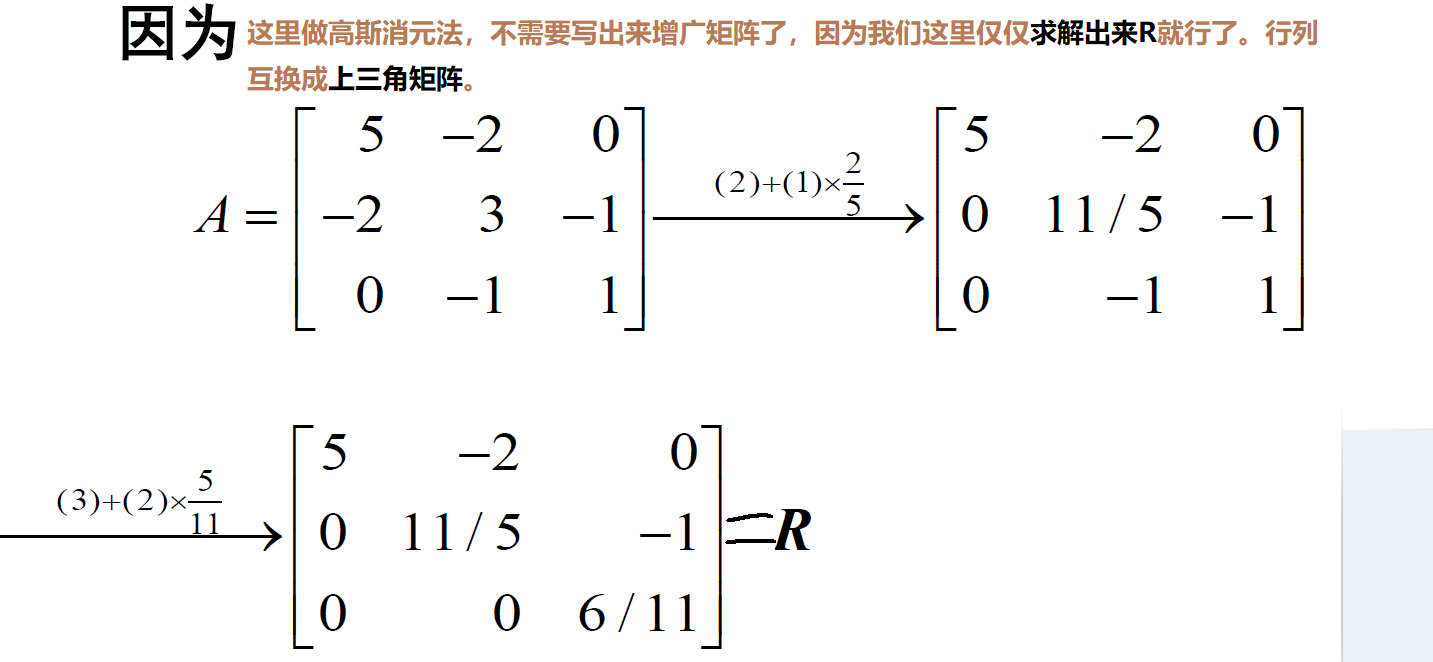然后把矩阵

R

\mathbf R

(上三角矩阵)分解为对角矩阵和单位上三角矩阵，就是进行LDR分解的一部分。

R

=

[

5

−

2

0

0

11

/

5

−

1

0

0

6

/

11

]

=

[

5

0

0

0

11

/

5

0

0

0

6

/

11

]

[

1

−

2

/

5

0

0

1

−

5

/

11

0

0

1

]

\mathbf R=\left[\begin{array}{ccc}{5} & {-2} & {0} \\ {0} & {11 / 5} & {-1} \\ {0} & {0} & {6 / 11}\end{array}\right]=\left[\begin{array}{ccc}{5} & {0} & {0} \\ {0} & {11 / 5} & {0} \\ {0} & {0} & {6 / 11}\end{array}\right]\left[\begin{array}{ccc}{1} & {-2 / 5} & {0} \\ {0} & {1} & {-5 / 11} \\ {0} & {0} & {1}\end{array}\right]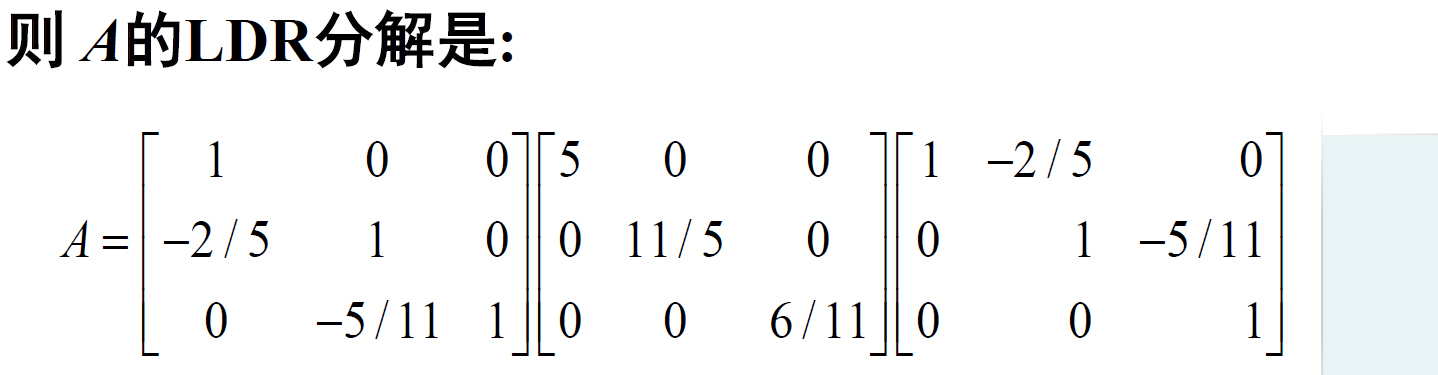注意：这里

L

\mathbf L

为：

L

=

[

1

−

2

/

5

0

0

1

−

5

/

11

0

0

1

]

T

\mathbf L= \left[\begin{array}{rrr}{1} & {-2 / 5} & {0} \\ {0} & {1} & {-5 / 11} \\ {0} & {0} & {1}\end{array}\right] ^{T}

因此我们可以得到下三角矩阵

G

=

R

T

D

1

2

\mathbf G=\mathbf R^{T} \mathbf D^{\frac{1}{2}}

G

=

[

1

0

0

−

2

/

5

1

0

0

−

5

/

11

1

]

[

5

0

0

0

11

/

5

0

0

0

6

/

11

]

=

[

5

0

0

−

2

/

5

11

/

5

0

0

−

5

/

11

6

/

11

]

\begin{aligned} \mathbf G &=\left[\begin{array}{ccc}{1} & {0} & {0} \\ {-2 / 5} & {1} & {0} \\ {0} & {-5 / 11} & {1}\end{array}\right]\left[\begin{array}{ccc}{\sqrt{5}} & {0} & {0} \\ {0} & {\sqrt{11 / 5}} & {0} \\ {0} & {0} & {\sqrt{6 / 11}}\end{array}\right] \\\\ &= \left[\begin{array}{ccc}{\sqrt{5}} & {0} & {0} \\ {-2 / \sqrt{5}} & {\sqrt{11 / 5}} & {0} \\ {0} & {-\sqrt{5 / 11}} & {\sqrt{6 / 11}}\end{array}\right] \end{aligned}

所以

A

\mathbf A

的平方根分解(Cholesky分解)为：

A

=

[

5

−

2

0

−

2

3

−

1

0

−

1

1

]

=

G

G

T

=

[

5

0

0

−

2

/

5

11

/

5

0

0

−

5

/

11

6

/

11

]

[

5

−

2

/

5

0

0

11

/

5

−

5

/

11

0

0

6

/

11

]

\mathbf A=\left[\begin{array}{ccc}{5} & {-2} & {0} \\ {-2} & {3} & {-1} \\ {0} & {-1} & {1}\end{array}\right]=\mathbf G \mathbf G^{\mathrm{T}}=\left[\begin{array}{ccc}{\sqrt{5}} & {0} & {0} \\ {-2 / \sqrt{5}} & {\sqrt{11 / 5}} & {0} \\ {0} & {-\sqrt{5 / 11}} & {\sqrt{6 / 11}}\end{array}\right]\left[\begin{array}{ccc}{\sqrt{5}} & {-2 / \sqrt{5}} & {0} \\ {0} & {\sqrt{11 / 5}} & {-\sqrt{5 / 11}} \\ {0} & {0} & {\sqrt{6 / 11}}\end{array}\right]

3.4. 平方根分解的求解之待定系数法
方法2：待定系数法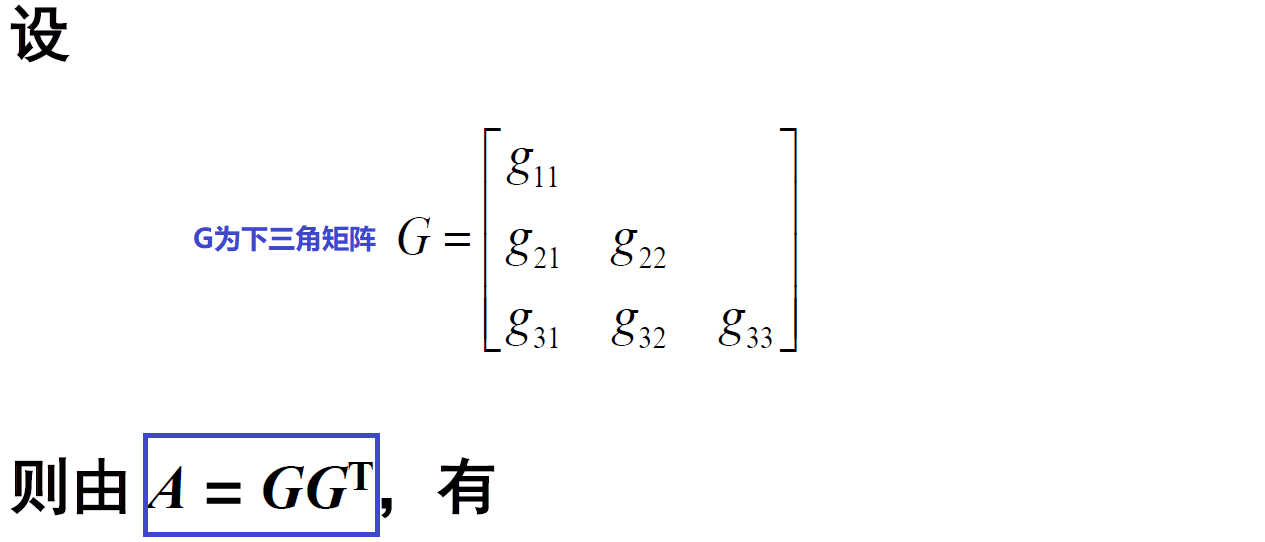我们可以得到如下的方程：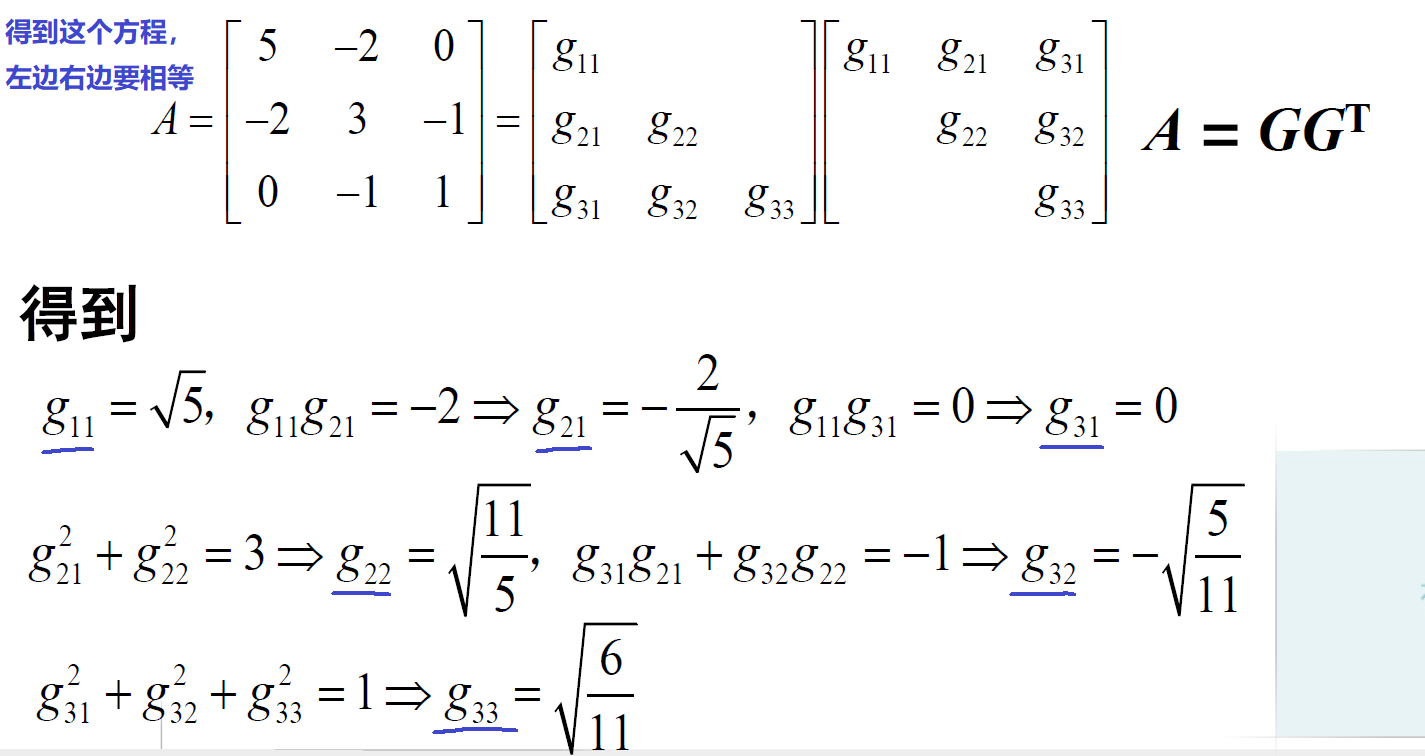所以

A

\mathbf A

的平方根分解(Cholesky分解)为：

A

=

[

5

−

2

0

−

2

3

−

1

0

−

1

1

]

=

G

G

T

=

[

5

0

0

−

2

/

5

11

/

5

0

0

−

5

/

11

6

/

11

]

[

5

−

2

/

5

0

0

11

/

5

−

5

/

11

0

0

6

/

11

]

\mathbf A=\left[\begin{array}{ccc}{5} & {-2} & {0} \\ {-2} & {3} & {-1} \\ {0} & {-1} & {1}\end{array}\right]=\mathbf G \mathbf G^{\mathrm{T}}=\left[\begin{array}{ccc}{\sqrt{5}} & {0} & {0} \\ {-2 / \sqrt{5}} & {\sqrt{11 / 5}} & {0} \\ {0} & {-\sqrt{5 / 11}} & {\sqrt{6 / 11}}\end{array}\right]\left[\begin{array}{ccc}{\sqrt{5}} & {-2 / \sqrt{5}} & {0} \\ {0} & {\sqrt{11 / 5}} & {-\sqrt{5 / 11}} \\ {0} & {0} & {\sqrt{6 / 11}}\end{array}\right]

四. Cholesky分解用在计算马氏距离
4.1. 马氏距离
马氏距离度量学习的核心是获得如下式所示的马氏距离函数：

d

M

2

(

x

,

z

)

=

(

x

−

z

)

T

M

(

x

−

z

)

d_{\boldsymbol M}^{2}(\boldsymbol{x}, \boldsymbol{z})=(\boldsymbol{x}-\boldsymbol{z})^{T} \boldsymbol{M}(\boldsymbol{x}-\boldsymbol{z})

其中

x

∈

R

n

\boldsymbol{x} \in \mathbb{R}^{n}

和

z

∈

R

n

\boldsymbol{z} \in \mathbb{R}^{n}

为

n

n

维特征空间中的

2

2

个样本，

M

⪰

0

\boldsymbol M \succeq 0

为一个

n

×

n

n × n

的对称半正定(Positive Semi-definite,PSD)矩阵。严格来说，马氏距离指代的是

d

M

(

x

,

z

)

=

(

x

−

z

)

T

M

(

x

−

z

)

d_{\boldsymbol M}(\boldsymbol{x}, \boldsymbol{z})=\sqrt{(\boldsymbol{x}-\boldsymbol{z})^{T} \boldsymbol{M}(\boldsymbol{x}-\boldsymbol{z})}

，这里实际上是马氏距离的平方。不过由于

M

\boldsymbol{M}

是一个半正定矩阵，所以

d

M

2

≥

0

d_{\boldsymbol M}^{2}\geq 0

。

d

M

d_{\boldsymbol M}

比

d

M

2

d_{\boldsymbol M}^2

多一次计算步骤而且对最终结果没有实质影响。因此一般将上述公式所示的函数称为马氏距离函数并用它来计算距离。同时由上述公式可知，在给定样本

x

\boldsymbol{x}

和

z

\boldsymbol{z}

之后，

d

M

2

(

x

,

z

)

d_{\boldsymbol M}^{2}(\boldsymbol{x}, \boldsymbol{z})

将由矩阵

M

\boldsymbol{M}

确定，即

d

M

2

(

x

,

z

)

d_{\boldsymbol M}^{2}(\boldsymbol{x}, \boldsymbol{z})

是由

M

\boldsymbol{M}

参数化的函数。文献中一般将矩阵

M

\boldsymbol{M}

称为度量矩阵，或简称为度量，马氏距离度量学习的核心任务即为获得具有判别性的矩阵

M

\boldsymbol{M}

。
对于

n

n

维特征空间中两个样本间的马氏距离

d

M

(

x

,

z

)

d_{\boldsymbol M}(\boldsymbol{x}, \boldsymbol{z})

，我们要求其需要满足以下的性质：

(1)

d

M

(

x

,

z

)

≥

0

(

距

离

的

非

负

性

)

\text { (1) } d_{\boldsymbol M}(\boldsymbol{x}, \boldsymbol{z}) \geq 0 (距离的非负性)

(2)

d

M

(

x

,

z

)

=

0

⟺

x

=

z

(

同

一

性

)

\text { (2) }d_{\boldsymbol M}(\boldsymbol{x}, \boldsymbol{z})=0 \Longleftrightarrow \boldsymbol{x}=\boldsymbol{z}(同一性)

(3)

d

M

(

x

,

z

)

=

d

M

(

z

,

x

)

(

距

离

的

对

称

性

)

\text { (3) }d_{\boldsymbol M}(\boldsymbol{x}, \boldsymbol{z})=d_{\boldsymbol M}(\boldsymbol{z}, \boldsymbol{x})(距离的对称性)

(4)

d

M

(

x

,

y

)

≤

d

M

(

x

,

z

)

+

d

M

(

z

,

y

)

(

距

离

三

角

不

等

式

)

\text { (4) }d_{\boldsymbol M}(\boldsymbol{x}, \boldsymbol{y}) \leq d_{\boldsymbol M}(\boldsymbol{x}, \boldsymbol{z})+d_{\boldsymbol M}(\boldsymbol{z}, \boldsymbol{y})(距离三角不等式)

为了使马氏距离函数满足上述各个性质，关键在于约束度量矩阵

M

\boldsymbol{M}

的半正定性，因此在马氏距离学习方法中一般都明确约束

M

⪰

0

\boldsymbol M \succeq 0

。另外，马氏距离与欧氏距离也存在密切的联系，如果对度量矩阵

M

\boldsymbol{M}

作 Cholesky分解有

M

=

L

L

T

\boldsymbol M=\boldsymbol L \boldsymbol L^{T}

(

L

(\boldsymbol L

为下三角矩阵

)

)

d

M

2

(

x

,

z

)

=

(

x

−

z

)

T

M

(

x

−

z

)

=

tr

⁡

(

M

T

(

x

−

z

)

(

x

−

z

)

T

)

=

(

x

−

z

)

T

L

L

T

(

x

−

z

)

=

(

L

T

(

x

−

z

)

)

T

L

T

(

x

−

z

)

\begin{aligned} d_{\boldsymbol M}^{2}(\boldsymbol{x}, \boldsymbol{z}) &=(\boldsymbol{x}-\boldsymbol{z})^{T} \boldsymbol{M}(\boldsymbol{x}-\boldsymbol{z}) \\&=\operatorname{tr}\left(\boldsymbol M^{T}(\boldsymbol{x}-\boldsymbol{z})(\boldsymbol{x}-\boldsymbol{z})^{T}\right) \\ &=(\boldsymbol{x}-\boldsymbol{z})^{T} \boldsymbol L \boldsymbol L^{T}(\boldsymbol{x}-\boldsymbol{z}) \\ &=\left(\boldsymbol{L}^{T}(\boldsymbol{x}-\boldsymbol{z})\right)^{T} \boldsymbol{L}^{T}(\boldsymbol{x}-\boldsymbol{z}) \end{aligned}

令

G

=

L

T

\boldsymbol G=\boldsymbol{L}^{T}

，则上面的等式可以变为如下：

d

M

2

(

x

,

z

)

=

[

G

(

x

−

z

)

]

T

[

G

(

x

−

z

)

]

=

∥

G

(

x

−

z

)

∥

2

2

\begin{aligned} d_{\boldsymbol M}^{2}(\boldsymbol{x}, \boldsymbol{z}) &=\left[\boldsymbol{G}(\boldsymbol{x}-\boldsymbol{z})\right]^{T} \left[\boldsymbol G(\boldsymbol{x}-\boldsymbol{z}) \right] \\ &=\left\|\boldsymbol{G}\left(\boldsymbol{x}-\boldsymbol{z}\right)\right\|_{2}^{2} \end{aligned}

即两个特征表达向量间的马氏距离等价于使用

G

\boldsymbol G

投影后的欧氏距离。当

M

\boldsymbol{M}

为单位矩阵时，马氏距离就转化成了欧氏距离

d

I

2

(

x

i

,

z

j

)

=

∥

x

i

−

z

j

∥

2

2

d_{\boldsymbol I}^{2}\left(\boldsymbol{x}_{i}, \boldsymbol{z}_{j}\right)=\left\|\boldsymbol{x}_{i}-\boldsymbol{z}_{j}\right\|_{2}^{2}

，但此时只能获得样本相同维度间的关系。
4.2. matlab实现计算马氏距离
测试样本971个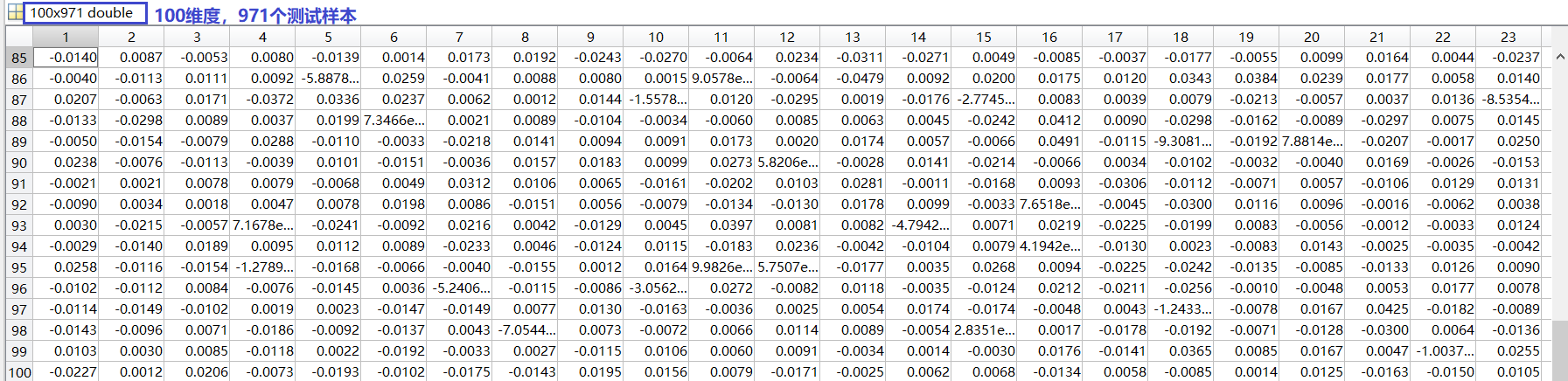训练样本2913个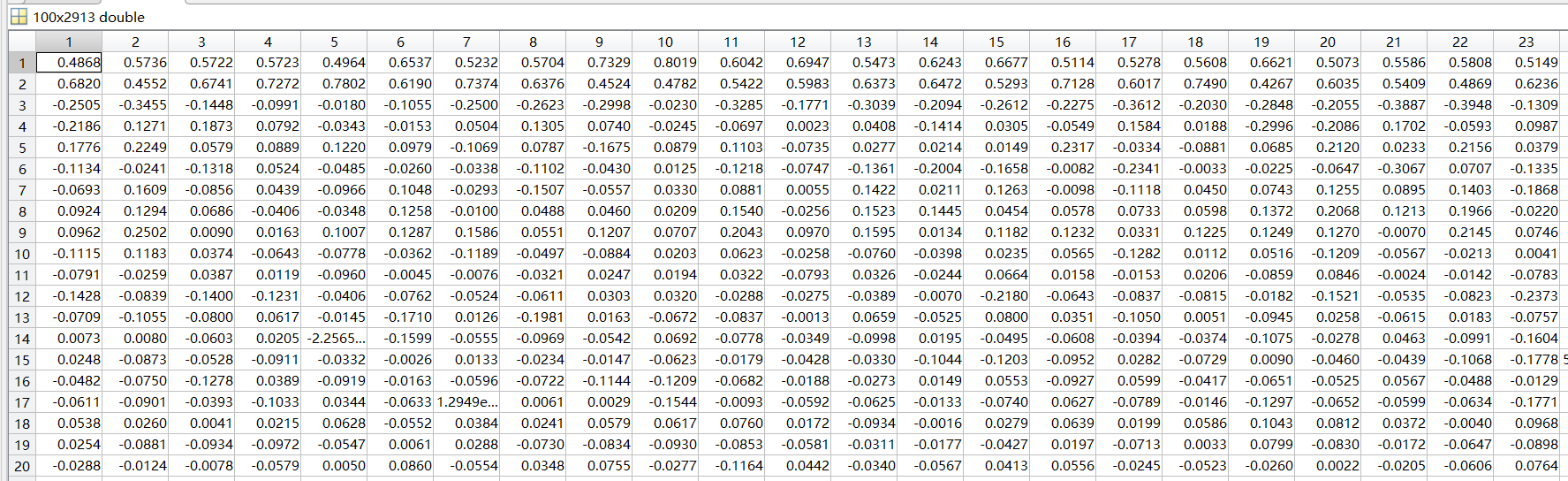假设这个时候已经更新出来度量矩阵

M

100

×

100

\boldsymbol{M_{100×100}}

，则可以计算马氏距离，我们可以轻易的发现计算出来的矩阵维度应该是

2913

×

971

2913×971

function dist = MahDist(M, Xg, Xp)
% function dist = MahDist(M, Xg, Xp)
% Mahalanobis distance
Xg = Xg';
Xp = Xp';
% Input:
%   <M>: the metric kernel
%   <Xg>: features of the gallery samples. Size: [n, d]
%   [Xp]: features of the probe samples. Optional. Size: [m, d]
%
%   Note: MahDist(M, Xg) is the same as MahDist(M, Xg, Xg).
%
% Output:
%   dist: the computed distance matrix between Xg and Xp

if nargin == 2                              % nargin是用来判断输入变量个数的函数
D = Xg * M * Xg';
u = diag(D);
dist = bsxfun(@plus, u, u') - 2 * D;
else
u = sum((Xg * M) .* Xg, 2);
v = sum((Xp * M) .* Xp, 2);
dist = bsxfun(@plus, u, v') - 2 * Xg * M * Xp';
end


最终计算出来的马氏距离：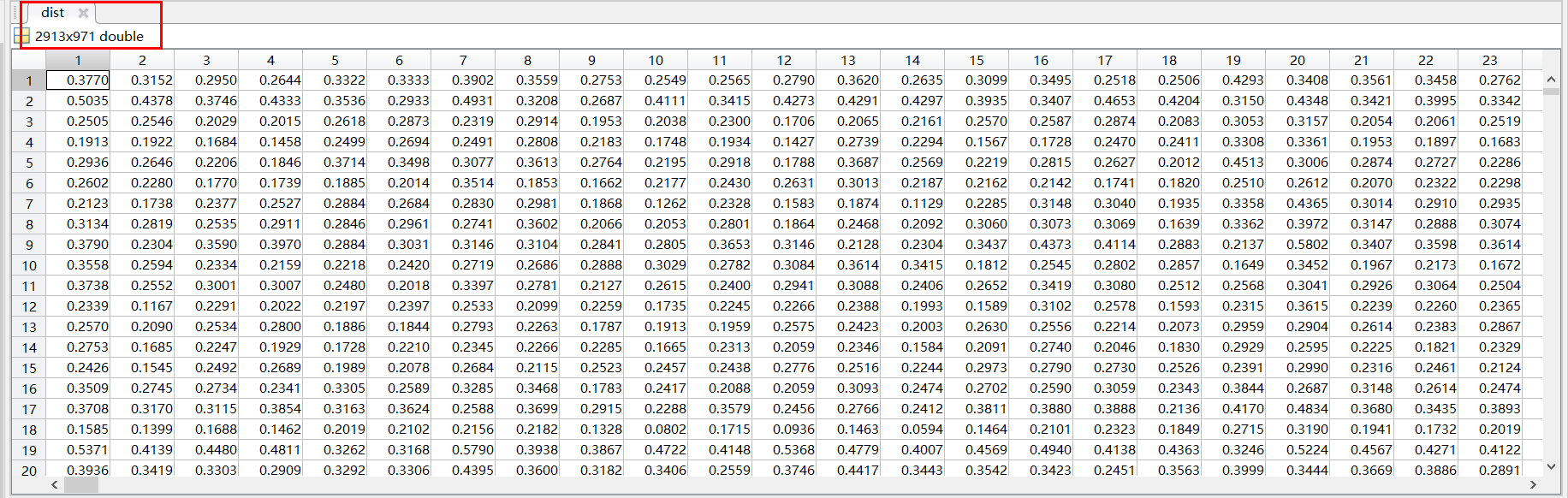matlab学习笔记 bsxfun函数；从计算时间上来说前两种实现差不多，远高于for的实现。但如果数据很大，第二种实现可能会有内存上的问题。所以bsxfun最好。

参考文献
本文主要参考国防科技大学杨文强教授课程PPT资料，这里表示感谢！
展开全文矩阵三角分解 Cholesky 马氏距离 平方根分解
• LR 分解 (Doolittle 分解) A=LR A=LR A=LR 其中，L 是单位下三角矩阵, R 是上三角矩阵 设 A 有 LR 分解： 比较矩阵两边元素，由第一行元素相等和第一列元素分别相等，可以得到： 按照第 1 行 + 第 1 列 → 第 2 行 ...
LR 分解 (Doolittle 分解)

A

=

L

R

A=LR

其中，L 是单位下三角矩阵, R 是上三角矩阵 设 A 有 LR 分解：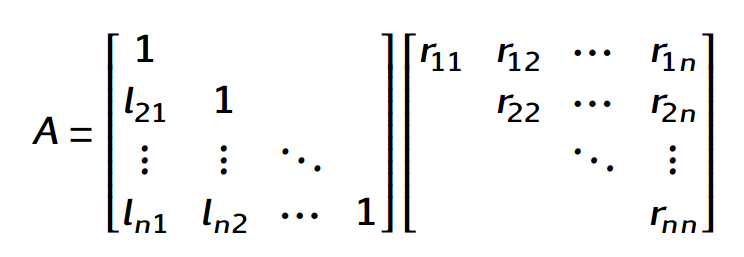比较矩阵两边元素，由第一行元素相等和第一列元素分别相等，可以得到：按照第 1 行 + 第 1 列 → 第 2 行 + 第 2 列 →…的顺序类推可以得到： 对于

i

=

1

,

.

.

.

,

n

i=1,...,n

，有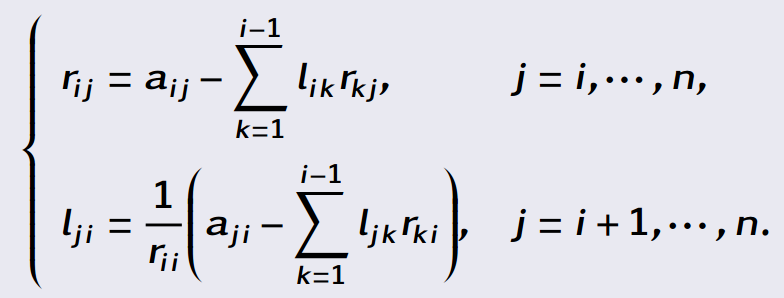LR 分解matlab程序
function[L_matrix,R_matrix] = LR_1(A_matrix)
[row_A,col_A] = size(A_matrix);

% 判断是否是非奇异方阵
if row_A ~= col_A
error('矩阵非方阵，无法进行LR分解');
end
if row_A ~= rank(A_matrix)
error('矩阵奇异，无法进行LR分解');
end

% 初始化
L_matrix = zeros(size(A_matrix));
R_matrix = zeros(size(A_matrix));

for j = 1:col_A
R_matrix(1,j) = A_matrix(1,j);
end
for i = 1:col_A
L_matrix(i,i) = 1;
end
for i = 2:row_A
L_matrix(i,1) = A_matrix(i,1) / A_matrix(1,1);
end

for i = 1:row_A
for j = i:row_A
sum = 0;
for k = 1:i-1
sum = sum + L_matrix(i,k) * R_matrix(k,j);
end
R_matrix(i,j) = A_matrix(i,j) - sum;
sum_1=0;
for k = 1:i-1
sum_1 = sum_1 + L_matrix(j,k) * R_matrix(k,i);
end
L_matrix(j,i) = (A_matrix(j,i) - sum_1) / R_matrix(i,i);
end
end


节约储存的LR分解 将 A 进行 LR 分解后，不再储存原来的 A ，直接将分解的 L 和 R 按照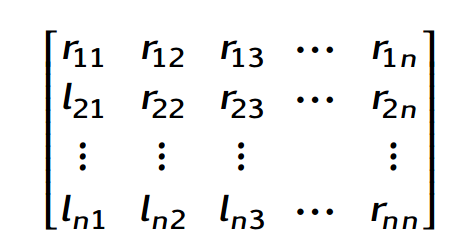这样的方式来存放，存放于矩阵 A 中，得到算法： 对于

i

=

1

,

.

.

.

,

n

i=1,...,n

，有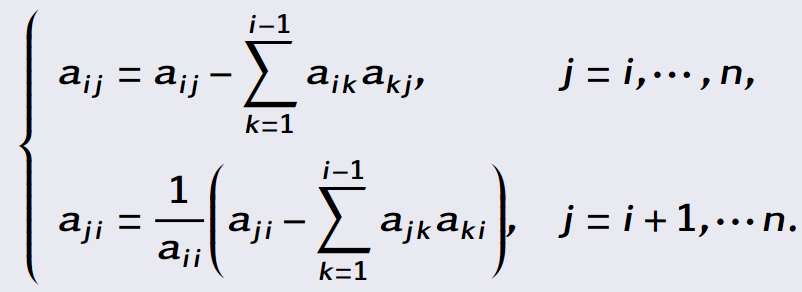function[A_matrix] = LR_2(A_matrix)
% 节省存储空间的 LR 分解算法
row_A = size(A_matrix,1);
for i = 1:row_A
T = det(A_matrix(1:i,1:i));
if T == 0
error('矩阵的顺序主子式为零，无法进行LR分解');
else
for j = i:row_A
sum = 0;
for k = 1:i-1
sum = sum+A_matrix(i,k) * A_matrix(k,j);
end
A_matrix(i,j) = A_matrix(i,j) - sum;
end
for j = i + 1:row_A
sum_1 = 0;
for k = 1:i-1
sum_1 = sum_1 + A_matrix(j,k) * A_matrix(k,i);
end
A_matrix(j,i) = (A_matrix(j,i) - sum_1) / A_matrix(i,i);
end
end
end


LDR分解

A

=

L

D

R

A=LDR

其中，L 是单位下三角矩阵, D 是对角矩阵, R 是单位上三角矩阵 可以直接在 LR 分解的基础上进行，

A

=

L

R

=

L

D

D

−

1

R

=

L

D

R

~

A=LR=LDD^{-1}R=LD\widetilde{R}

其中， D 即为 LR 分解中的 R 的对角线元素组成的矩阵，

D

=

d

i

a

g

(

r

11

,

r

22

,

.

.

.

r

n

n

)

D=diag(r_{11},r_{22},...r_{nn})

此时

R

~

\widetilde{R}

为单位上三角矩阵LDR分解matlab代码
function[L_matrix,D_matrix,R_matrix] = LDR_1(A_matrix)
[row_A,col_A] = size(A_matrix);

% 判断是否是非奇异方阵
if row_A ~= col_A
error('矩阵非方阵，无法进行LR分解');
end
if row_A ~= rank(A_matrix)
error('矩阵奇异，无法进行LR分解');
end

% 初始化
L_matrix = zeros(size(A_matrix));
D_matrix = zeros(size(A_matrix));
R_matrix = zeros(size(A_matrix));

% 先进行LR分解
for j = 1:col_A
R_matrix(1,j) = A_matrix(1,j);
end
for i = 1:col_A
L_matrix(i,i) = 1;
end
for i = 2:row_A
L_matrix(i,1) = A_matrix(i,1) / A_matrix(1,1);
end

for i = 1:row_A
for j = i:row_A
sum = 0;
for k = 1:i-1
sum = sum + L_matrix(i,k) * R_matrix(k,j);
end
R_matrix(i,j) = A_matrix(i,j) - sum;
sum_1=0;
for k = 1:i-1
sum_1 = sum_1 + L_matrix(j,k) * R_matrix(k,i);
end
L_matrix(j,i) = (A_matrix(j,i) - sum_1) / R_matrix(i,i);
end
end
% 进行完LR分解之后，将R分解为D*R
for i = 1:row_A
D_matrix(i,i) = R_matrix(i,i);
end
for i = 1:row_A
for j = 1:row_A
if i <= j
R_matrix(i,j) = R_matrix(i,j) / D_matrix(i,i);
end
end
end


节约储存的LDR分解matlab代码 同样可以得到节约存储的LDR分解，将 L 放入原来 A 矩阵的下三角， D 放入对角线，R 放入上三角
function[A_matrix]=LDR_2(A_matrix)
% 节省存储空间的 LDR 分解算法
row_A = size(A_matrix,1);

% 先进行节省存储空间的LR分解
for i = 1:row_A
T = det(A_matrix(1:i,1:i));
if T == 0
error('矩阵的顺序主子式为零，无法进行LR分解');
else
for j = i:row_A
sum = 0;
for k = 1:i-1
sum = sum+A_matrix(i,k) * A_matrix(k,j);
end
A_matrix(i,j) = A_matrix(i,j) - sum;
end
for j = i + 1:row_A
sum_1 = 0;
for k = 1:i-1
sum_1 = sum_1 + A_matrix(j,k) * A_matrix(k,i);
end
A_matrix(j,i) = (A_matrix(j,i)-sum_1) / A_matrix(i,i);
end
end
end

% 进行完LR分解之后，将R分解为D*R，用节省存储空间的方式储存
for i = 1:row_A
for j = 1:row_A
if i < j
A_matrix(i,j) = A_matrix(i,j) / A_matrix(i,i);
j=j+1;
else
A_matrix(i,j) = A_matrix(i,j);
end
end
end


矩阵的分解还有很多很多，这里空白太小,我写不下了 0.0
展开全文矩阵 matlab
•线性代数 LU分解
• 定义：给定矩阵A，将A表示成下三角矩阵L和上三角矩阵U的乘积，称为LU分解线性代数 算法
• ## SVD分解

千次阅读 2018-04-14 22:22:57
奇异值分解（Singular Value Decomposition，以下简称SVD）是矩阵分解中三大分解方法之一，另外2个为LR分解以及QR分解。 奇异值分解对矩A进行分解，得到了3个矩阵的乘积： 改：右奇异向量，是A^TA的正交特征...
• 目录简述矩阵分解定义作用三角分解LU分解（LR分解）QR分解奇异值分解（SVD分解）特征值分解（谱分解） 简述矩阵分解 定义 把一个矩阵表示为多个矩阵连乘的形式。 作用 用更少的内存消耗，存储一样多信息。eg：稀疏...
• 行业分类-设备装置-一种基于对称稀疏矩阵技术的改进LR三角分解求取电力系统节点阻抗矩阵的方法.zip
• 高斯消元法 首先判断顺序主子式 带行交换的LR分解 例子 待定系数法 。。。就是一个一个算矩阵论
• LU分解是很多算法的基础，就来我来轻轻松松的水一篇博文把。这是程序员的线性代数读书笔记——LU...LU分解也成为LR分解，LDU分解本质上也是LU分解 简单的说，LU分解给计算机的计算带来了很大的好处。 ...
• xLearn是一种高性能，易于使用且可扩展的机器学习程序包，其中包含线性模型（LR），因式分解机（FM）和现场感知的因式分解机（FFM），所有这些都可用于解决大型问题。规模的机器学习问题。 xLearn对于解决大规模...
• 上一篇谈了矩阵分解中比较简单的三角分解，这里介绍另外两种分解：矩阵的谱分解和LR分解。 矩阵的谱是指矩阵所有特征值的集合。因此，矩阵的谱分解就是利用矩阵的特征值来对矩阵分解。利用矩阵对角化可以得到：任何...
• %矩阵的LR分解 方阵A是非奇异的 clear; A = [2,1,4;4,3,13;2,2,20]; format rat [L,U]=lu(A) % [L,U,P]=lu(A) %% %矩阵QR分解 [Q,R]=qr(A） R为上三角矩阵 clear;clc A=[0 4 1;1 1 1;0 1 2]; [Q,R,e]=qr(A); %% %...matlab
• 本博客可能不会对分解讲的特别深入，主要是想弄清楚各个分解的条件、分解结果以及应用（或特点）。 包括： 1、三角分解（LU分解） 2、LDLT分解与LLT分解（Cholesky分解） 3、QR分解 4、奇异值分...
• 本博客主要介绍在SLAM问题中常常出现的一些线性代数相关的知识，重点是如何采用矩阵分解的方法，...包括：1、三角分解（LU分解）2、QR分解3、特征值分解4、奇异值分解（SVD分解）5、LDLT分解6、LLT分解（Cholesky分解最小二乘 SVDpython 机器学习 推荐系统
• 《编译原理》构造 LR(1) 分析表的步骤与例题解析
• 协同过滤： 源于1992年直到2003年才被Amazon发表论文...将协同过滤算法中的共现矩阵分解为用户矩阵和物品矩阵，利用用户因向量和物品因向量的内积进行排序并推荐 特点： 相较协同过滤，泛化能力有所加强，对稀疏矩深度学习 机器学习 推荐系统 人工智能 协同过滤
• （1）一个对角元素都是1的下三角矩阵，称为单位下三角矩阵。 （2）上（下）三角矩阵的乘积仍是上...三角分解如果方阵 AA 可分解为一个下三角矩阵 LL 和一个上三角矩阵 UU 的乘积，则称 AA 可作三角分解或 LU(LR)LU(LR)
• 在协同过滤和矩阵分解存在的劣势就是仅利用了用户与物品相互行为信息进行推荐， 忽视了用户自身特征， 物品自身特征以及上下文信息等，导致生成的结果往往会比较片面。 2014年由Facebook提出的GBDT+LR模型， 该模型...
• 1 为什么将LR和SVM放在一起来进行比较？ 回答这个问题其实就是回答LR和SVM有什么相同点。 第一，LR和SVM都是分类算法。 看到这里很多人就不会认同了，因为在很大一部分人眼里，LR是回归算法。我是非常不赞同这...svm 机器学习
• 今天讲解LR(0)SLR(1)LR(1) 首先是自底向上分析过程: 为一个输入串构造语法分析树的过程 LR(k)分析技术: L:从左向右 R: 反向构造一个最右推导序列 k: 做出语法分析决定时向前看k个输入符号 当然在实践中我们只考虑k...编译器...